# Selina Solutions Concise Maths Class 10 Chapter 5 Quadratic Equations

An equation with one variable, in which the highest power of the variable is two, is known as quadratic equation. Students who wish to develop a strong grip over the concepts of Selina can use Selina Solutions for this purpose. This resource can also be used by students for preparation of their exams too. All the solutions are prepared by subject experts at BYJU’S to match all levels of students.

Chapter 5 of Concise Selina for Class 10 Maths has six exercises. Understanding quadratic equations, examining the nature of the roots, solving quadratic equations by factorisation and quadratic formula are the key topics covered in this chapter. Also, students can make use of the Concise Selina Solutions for Class 10 Maths Chapter 5 Quadratic Equations PDF from the links given below.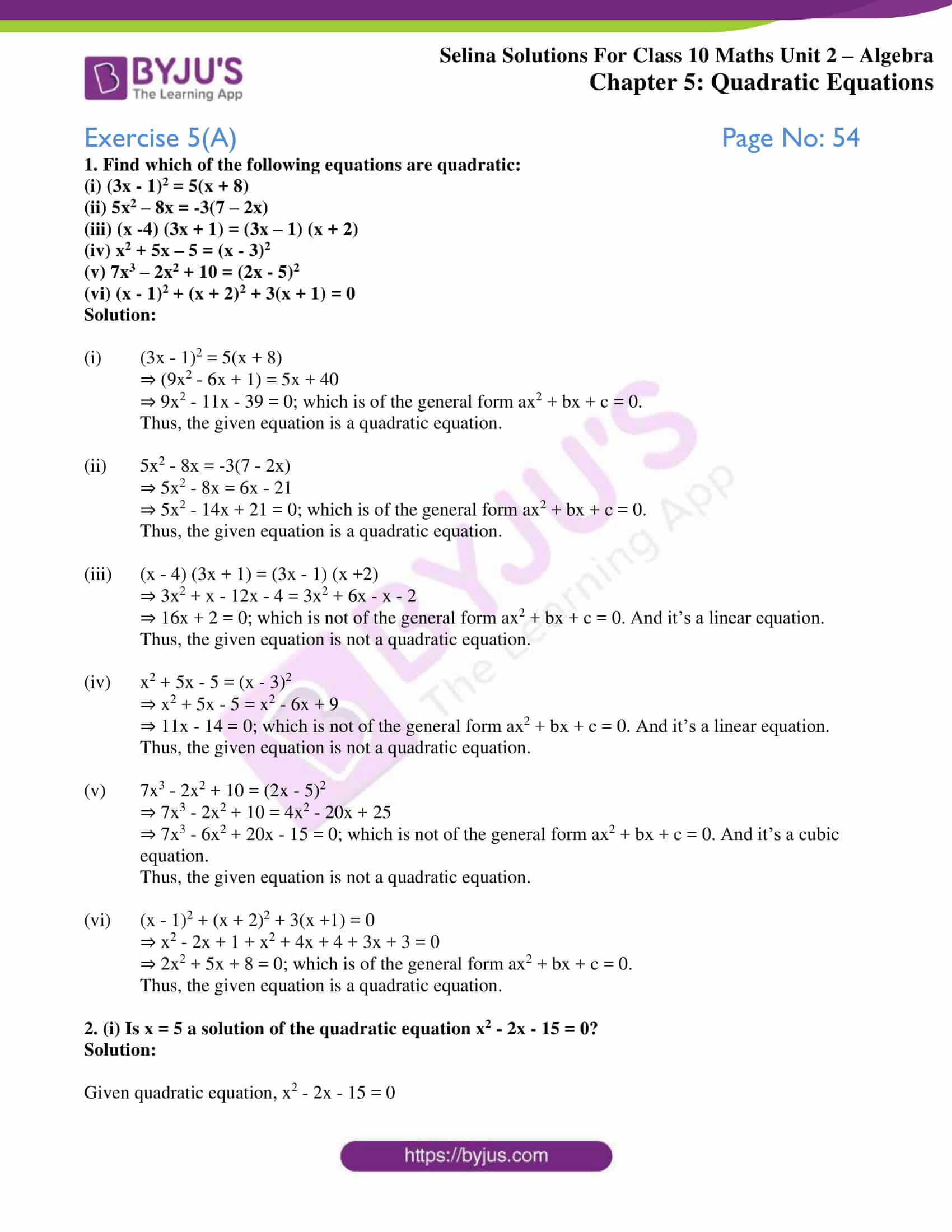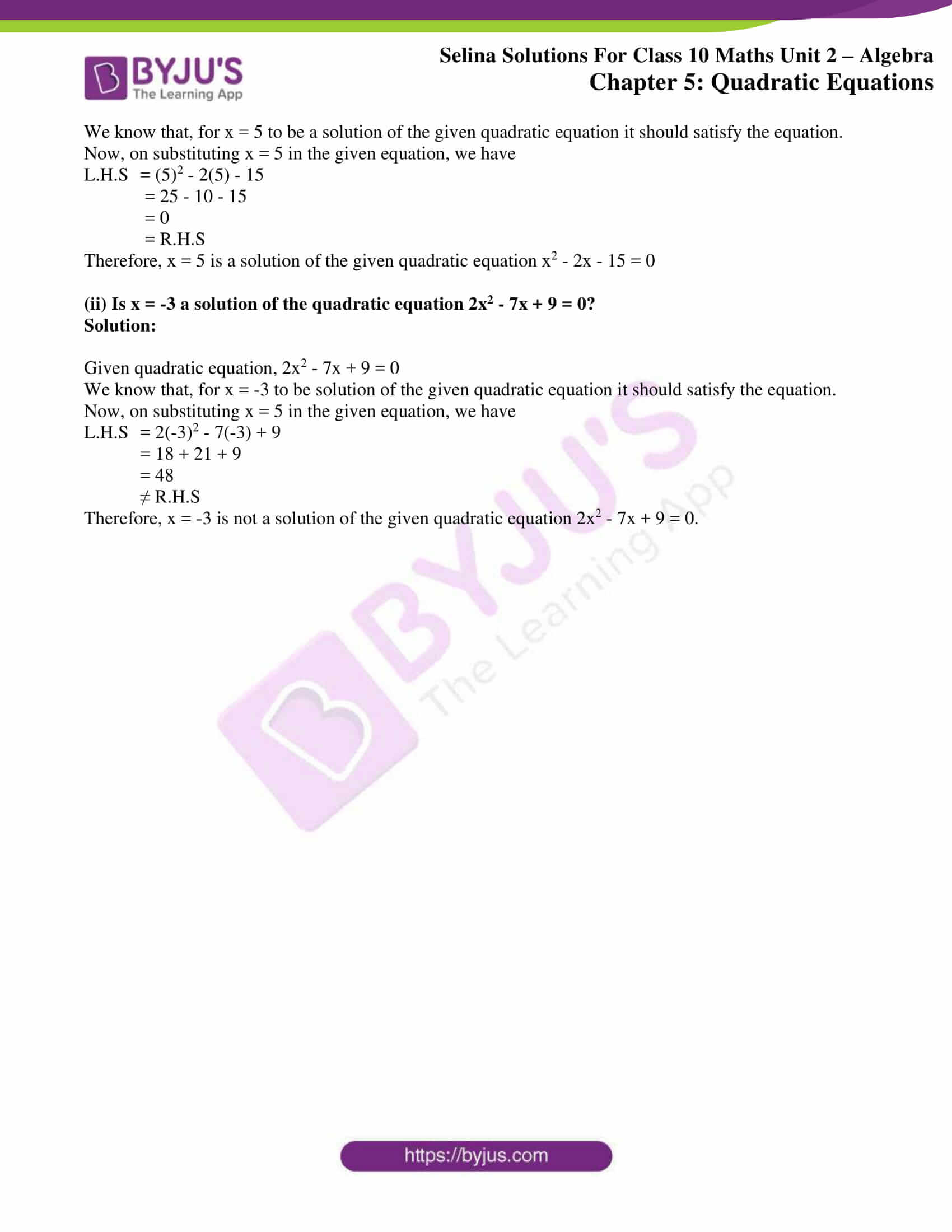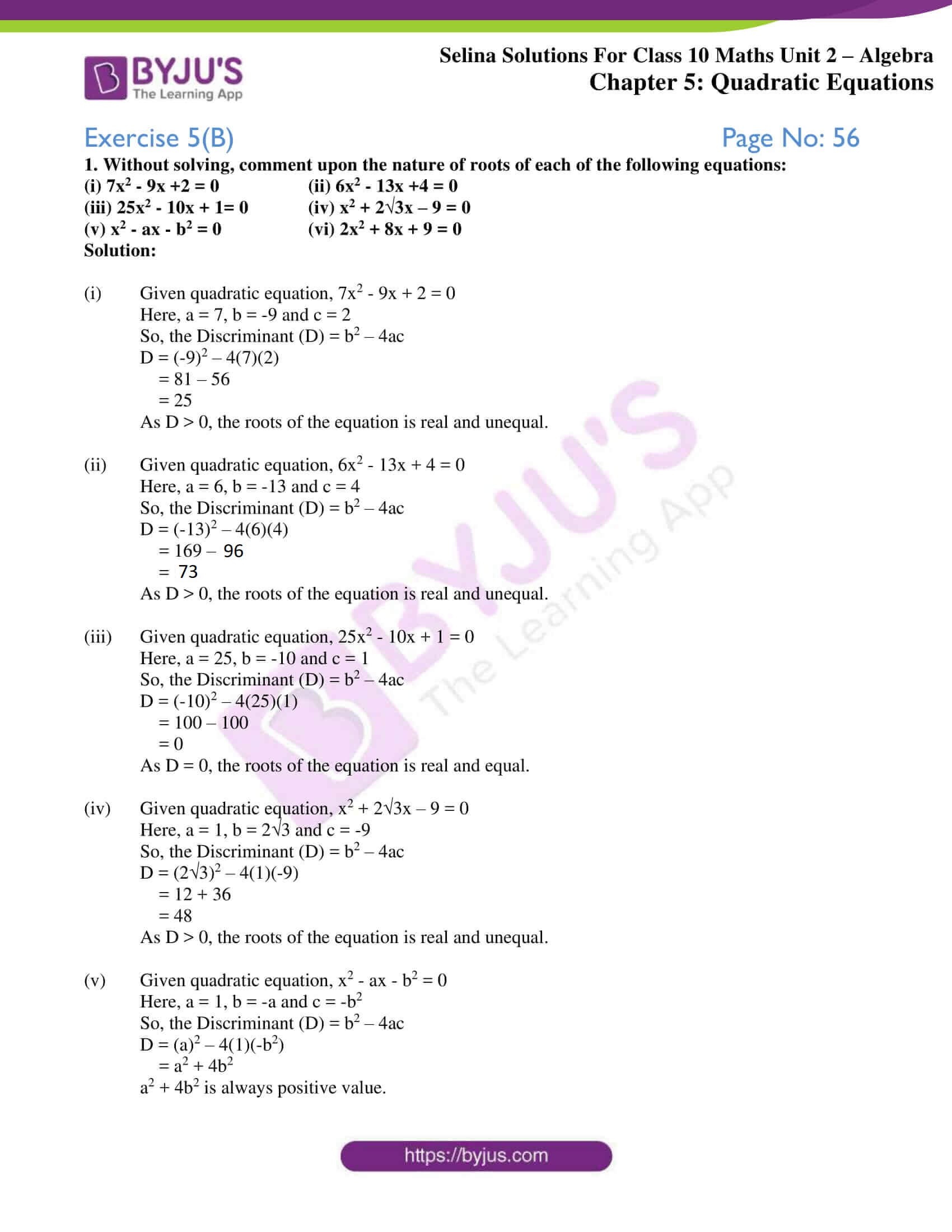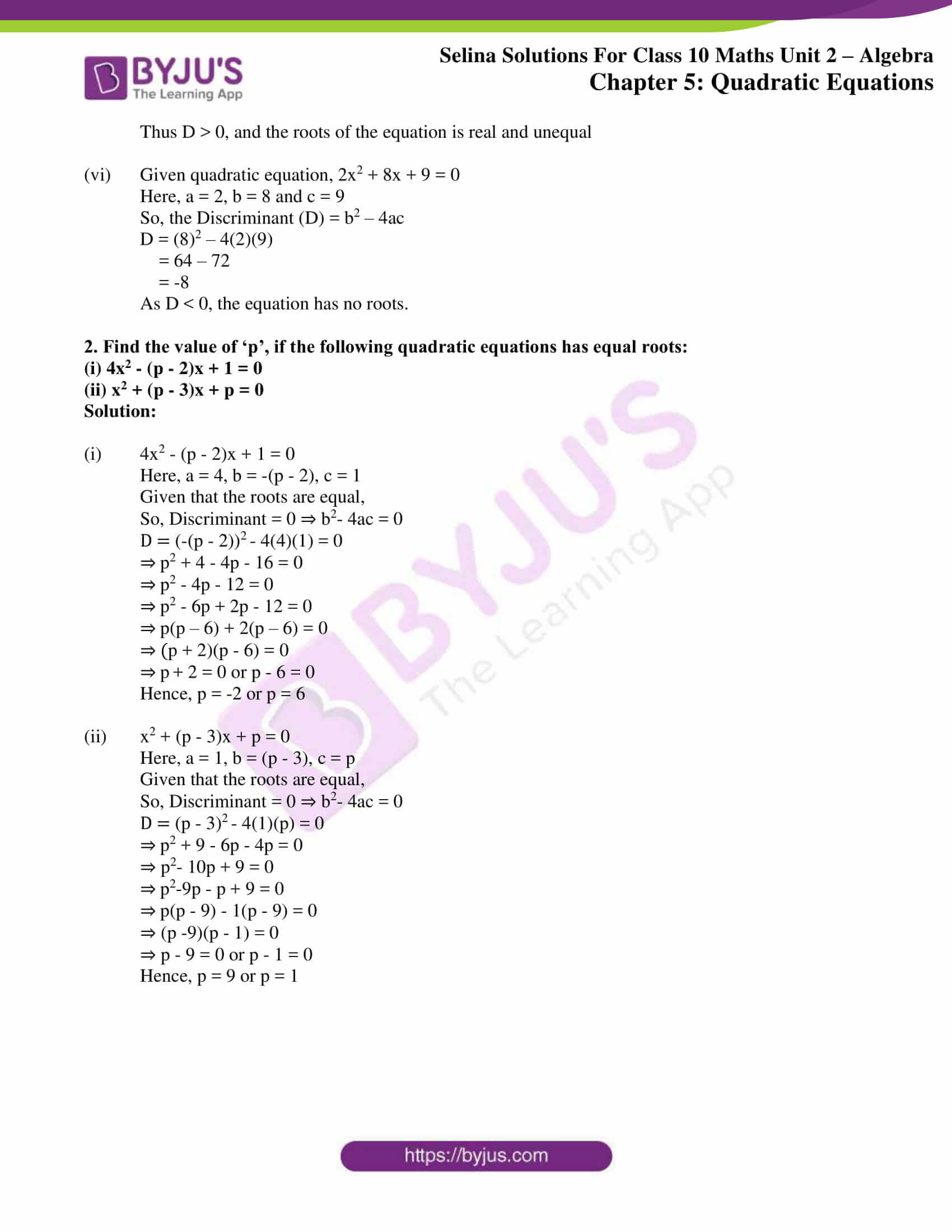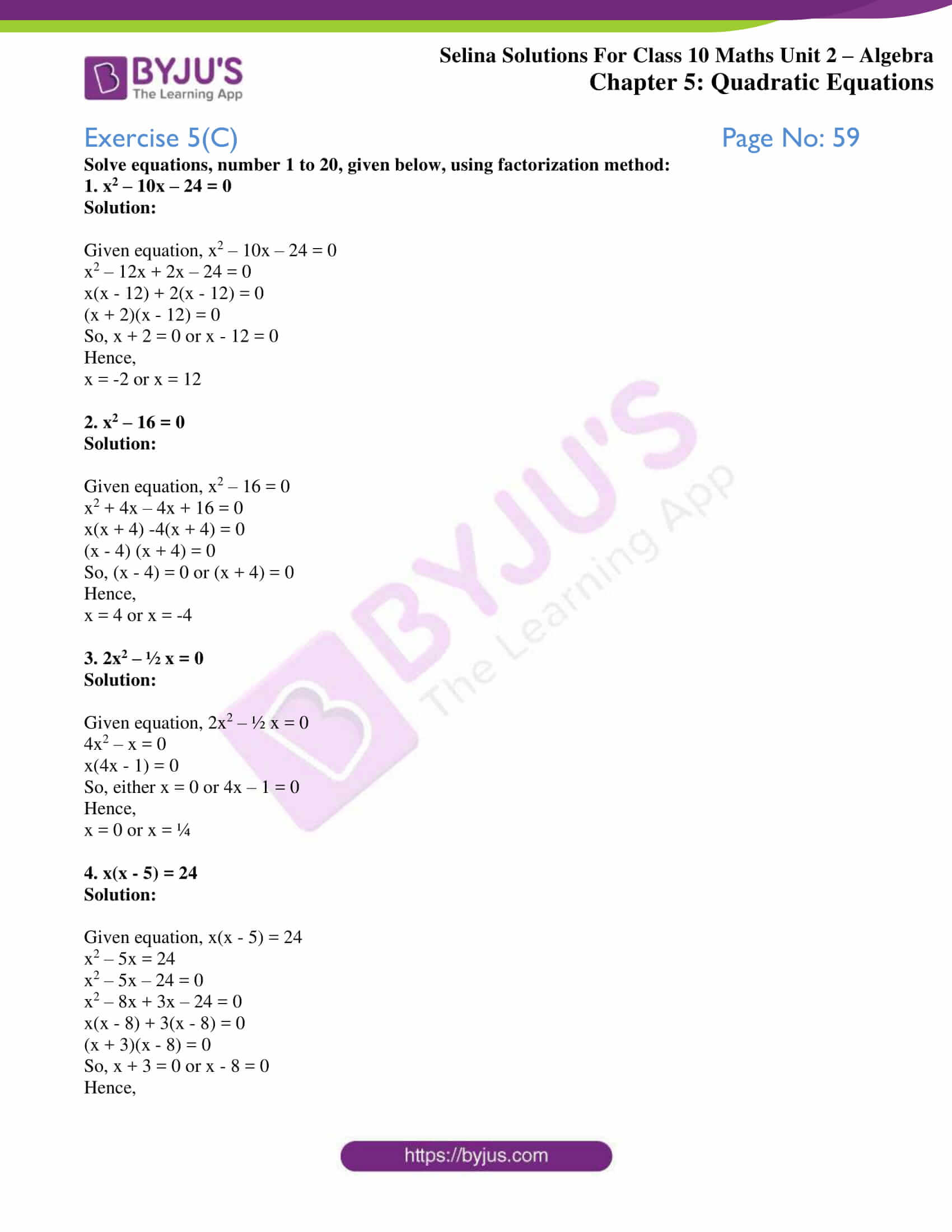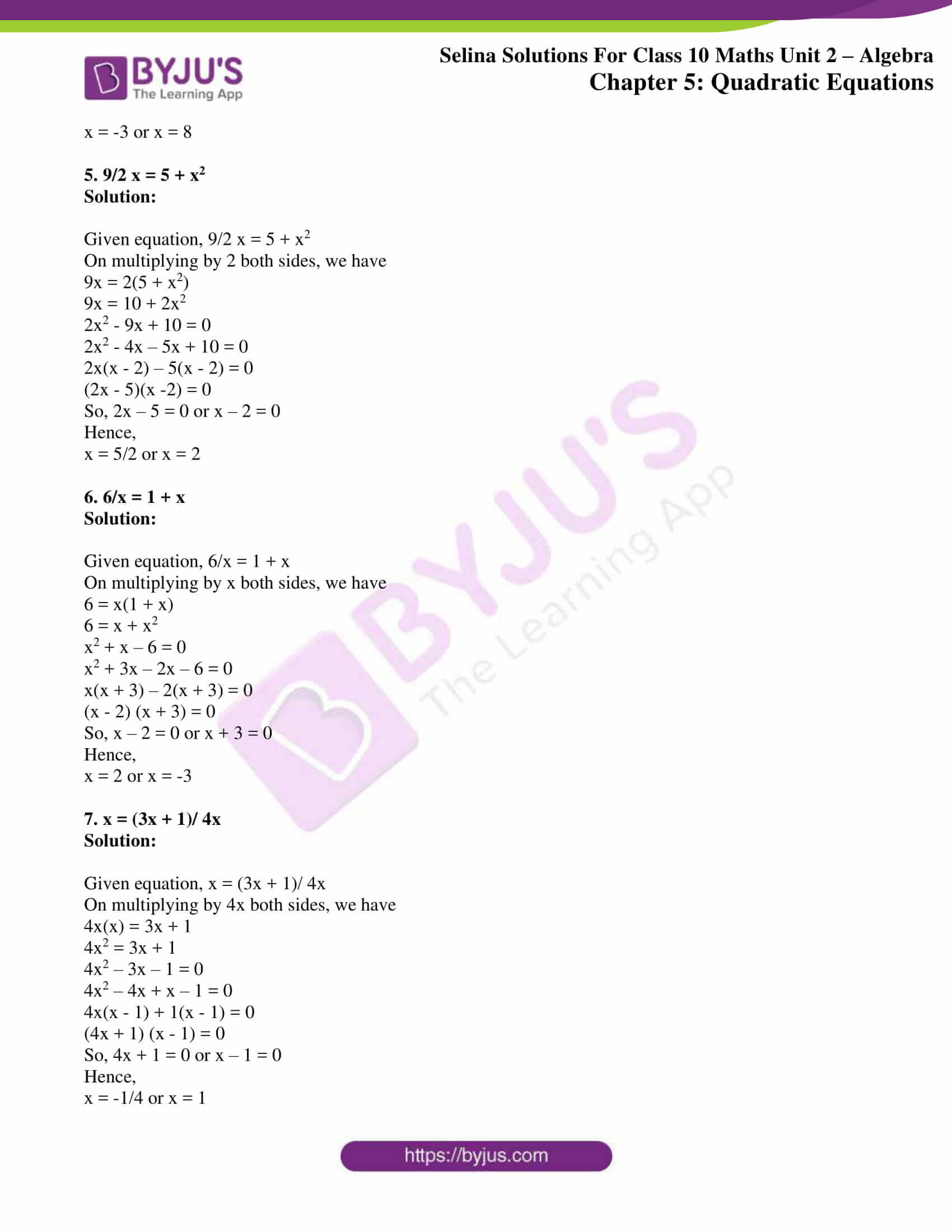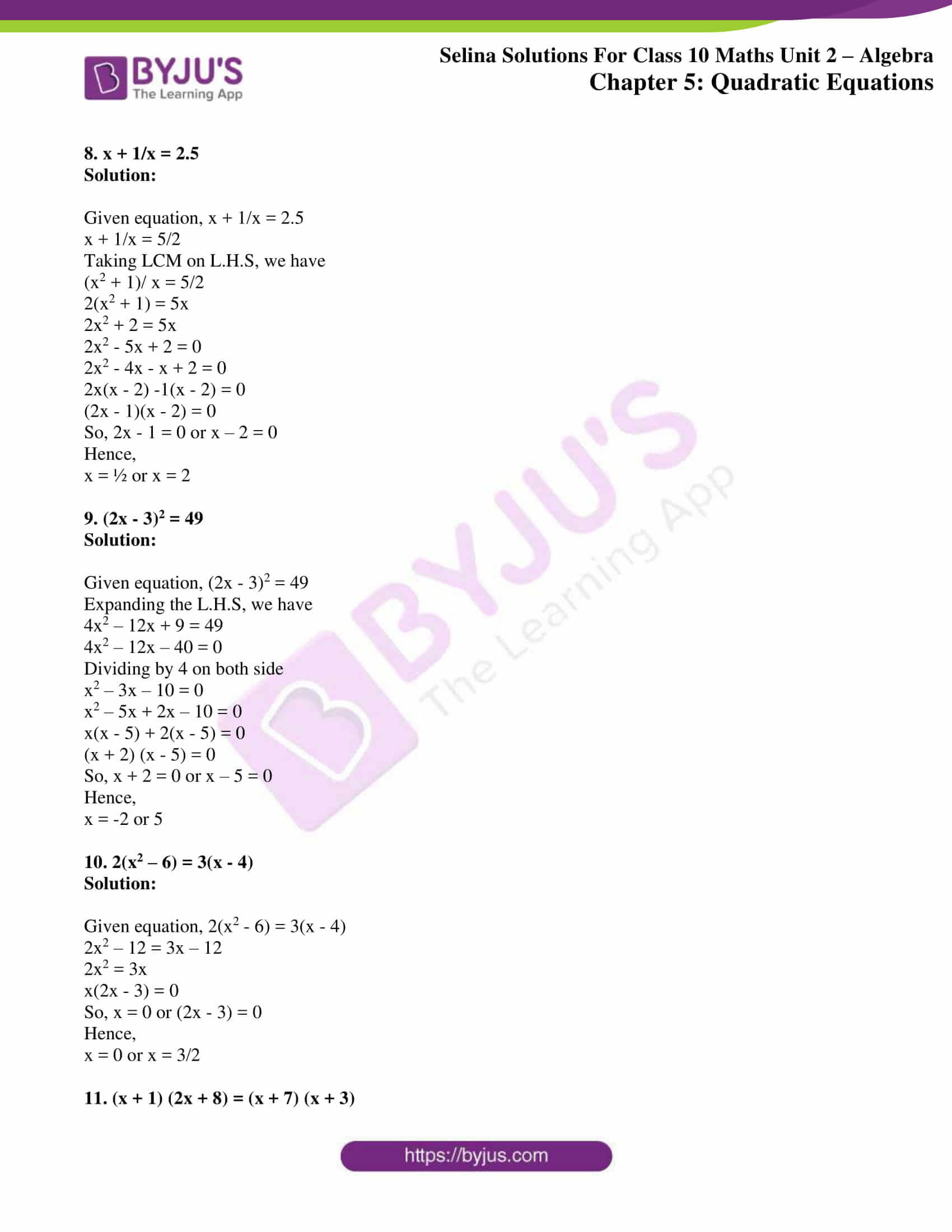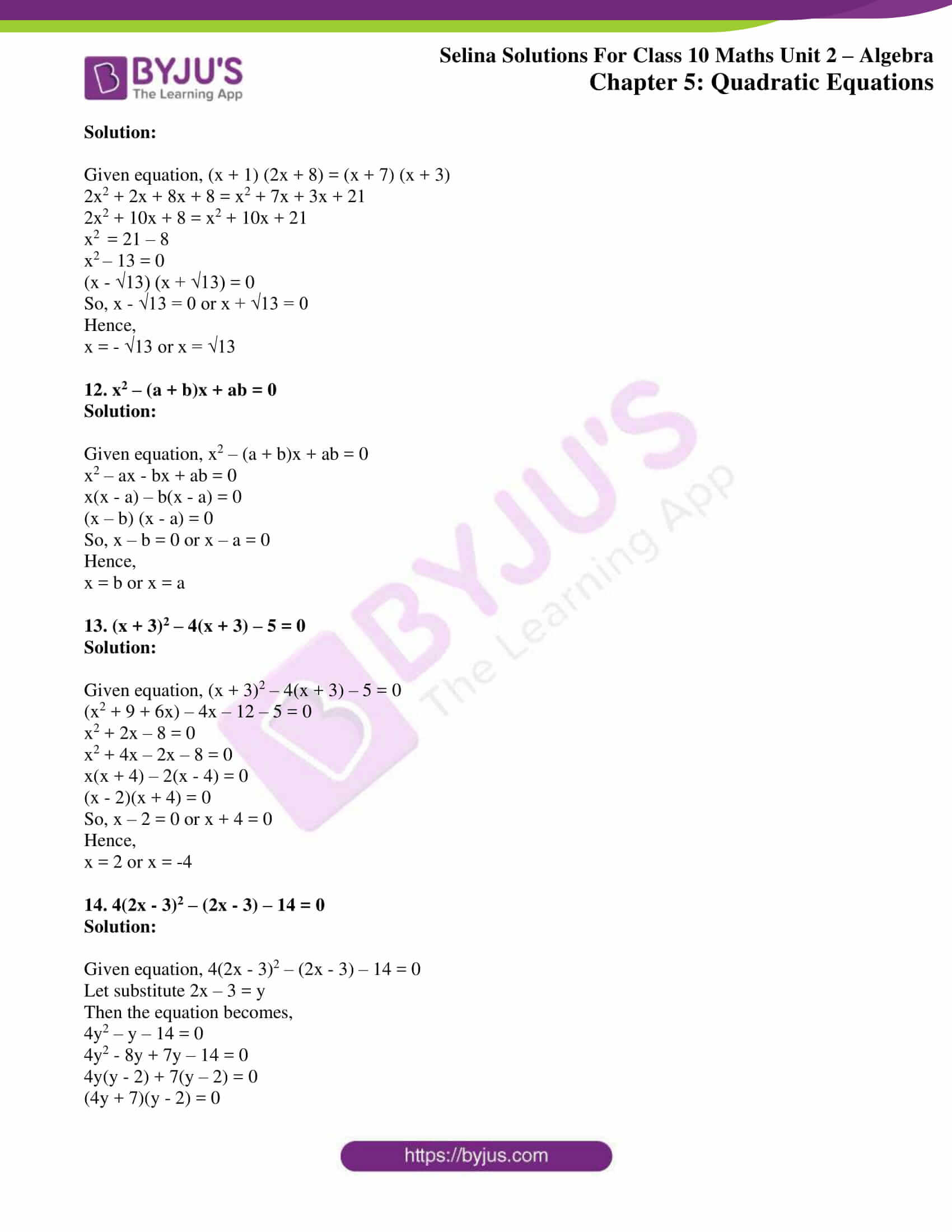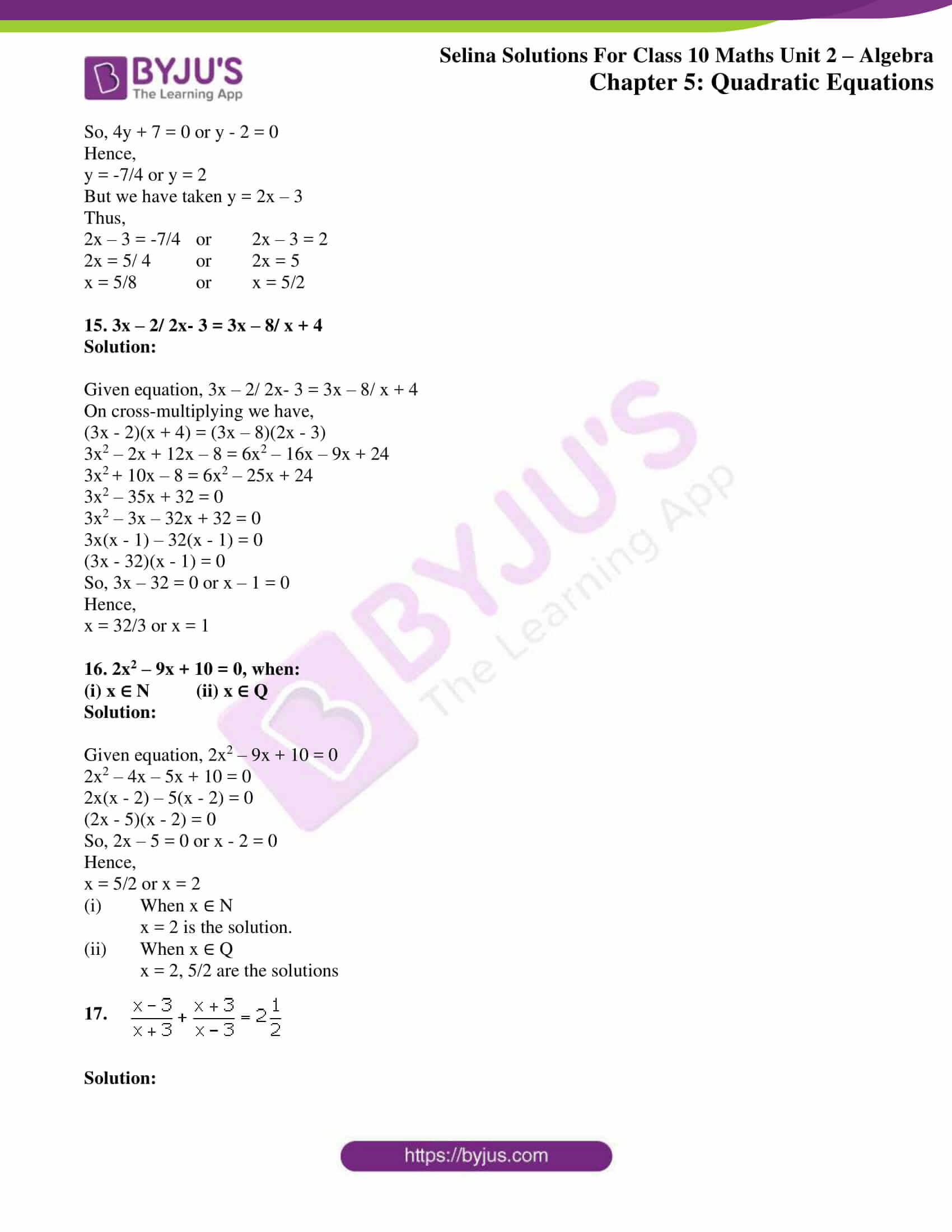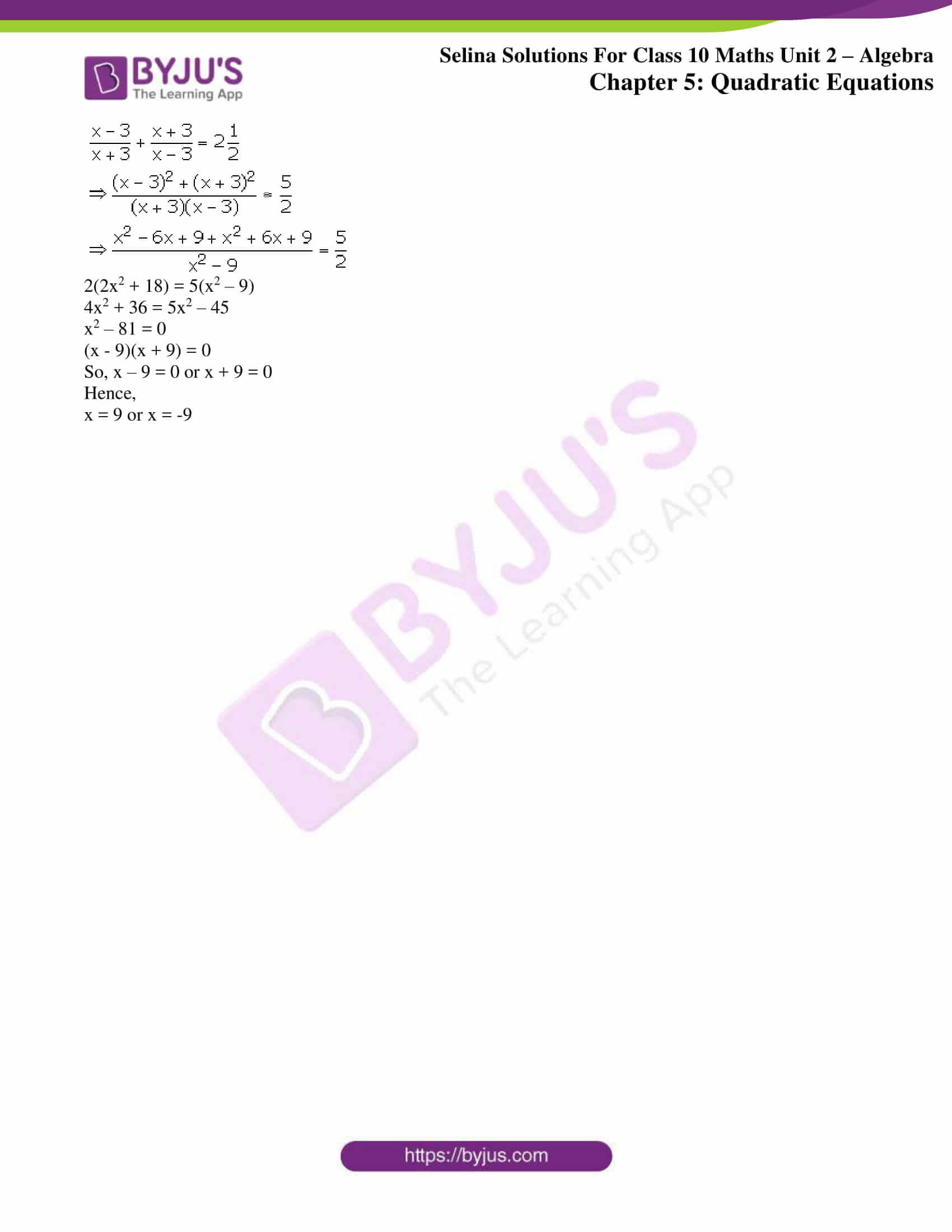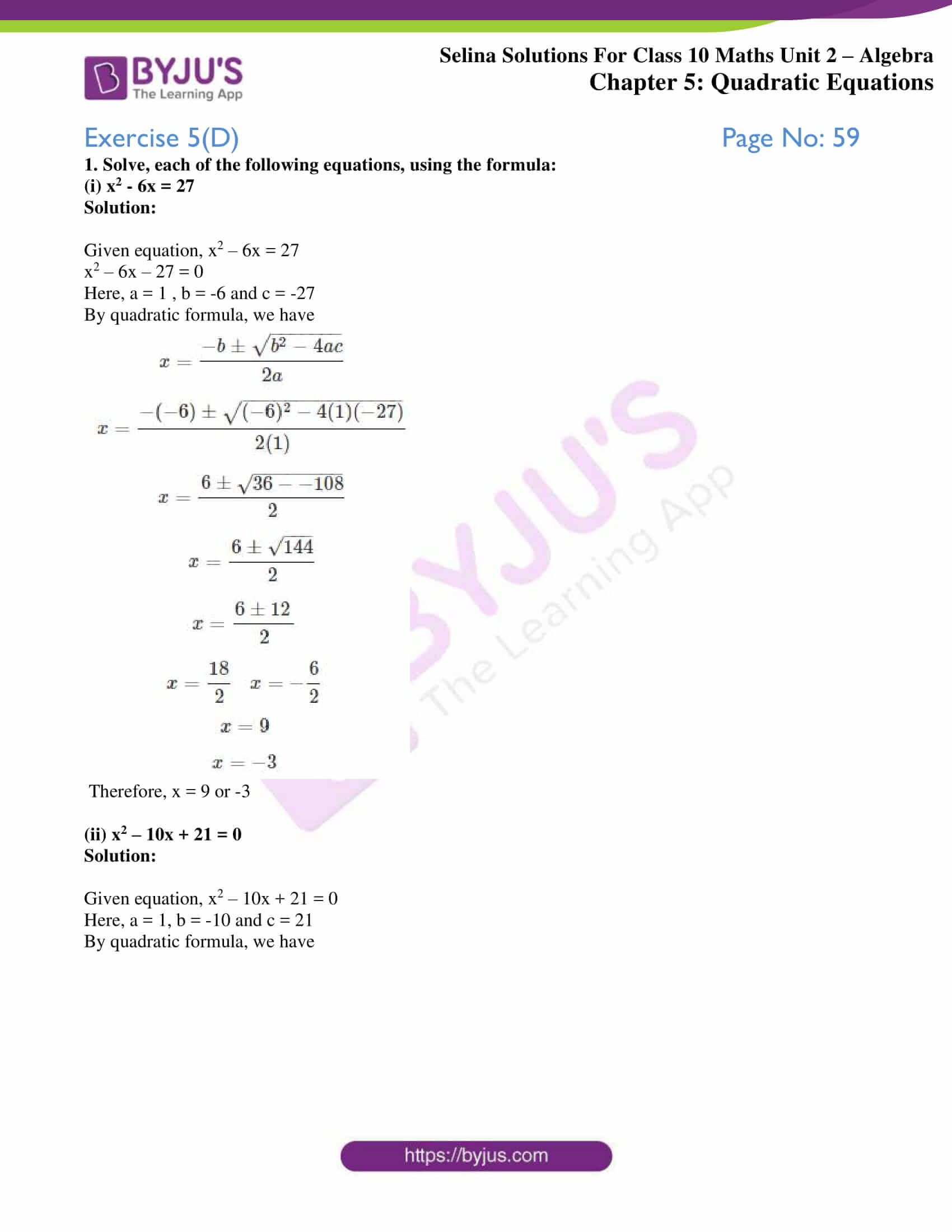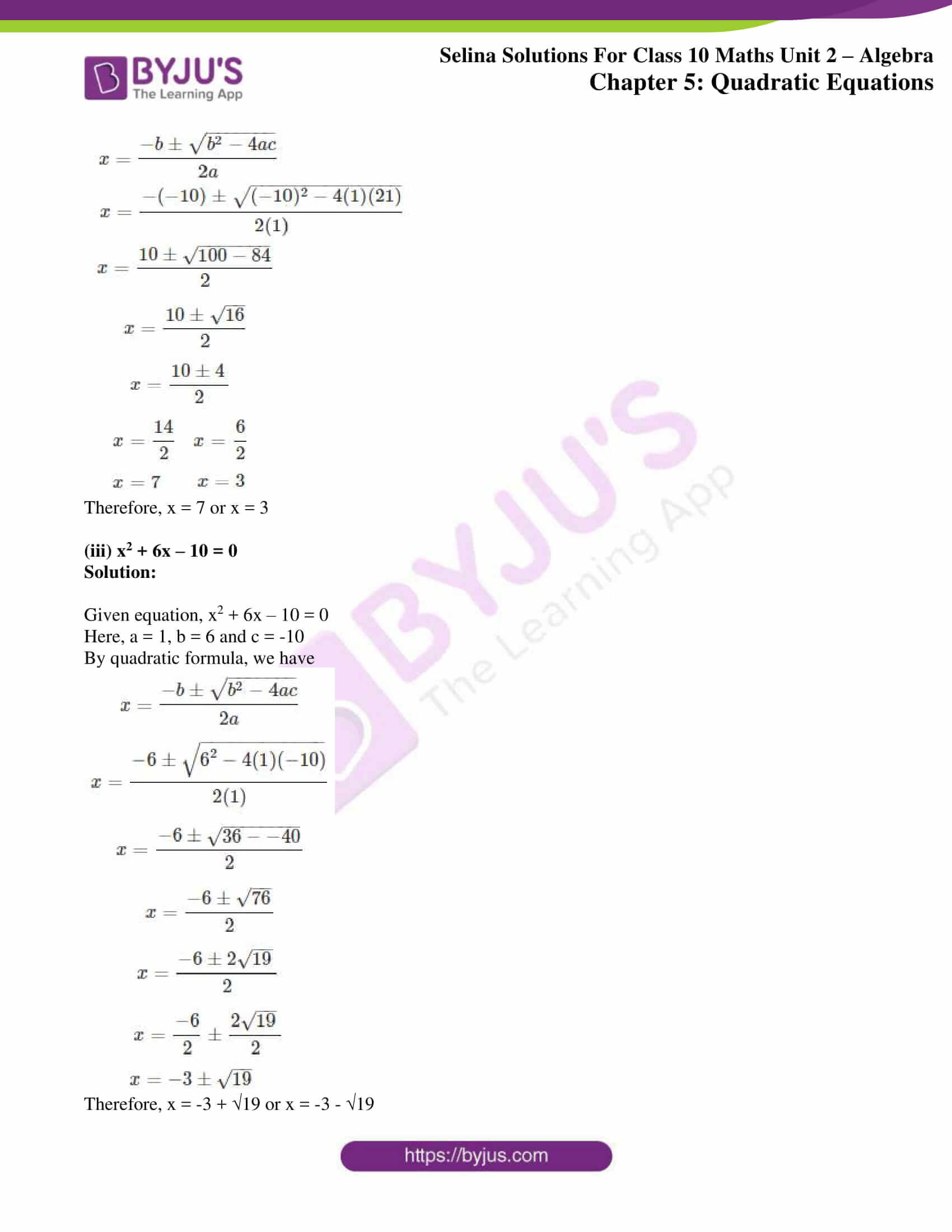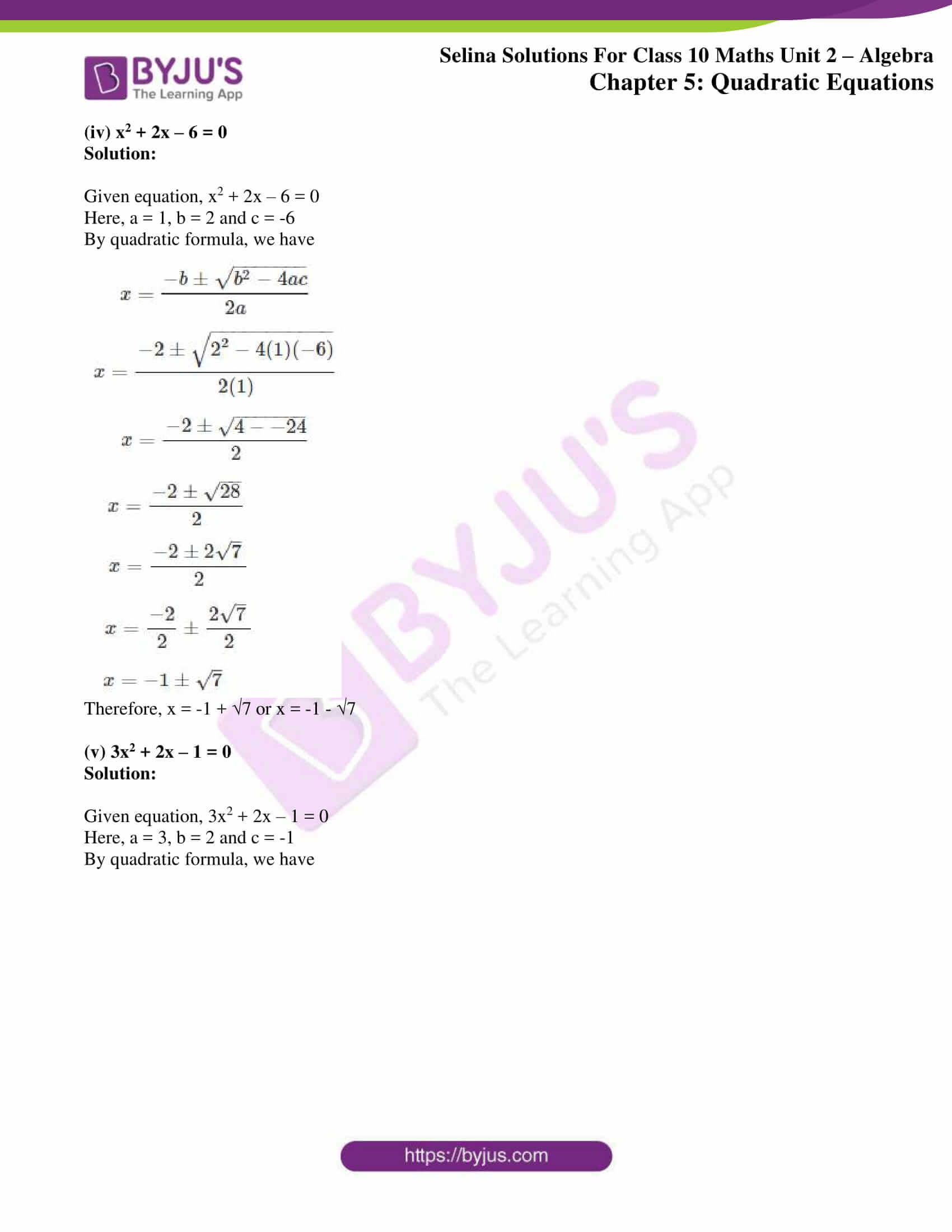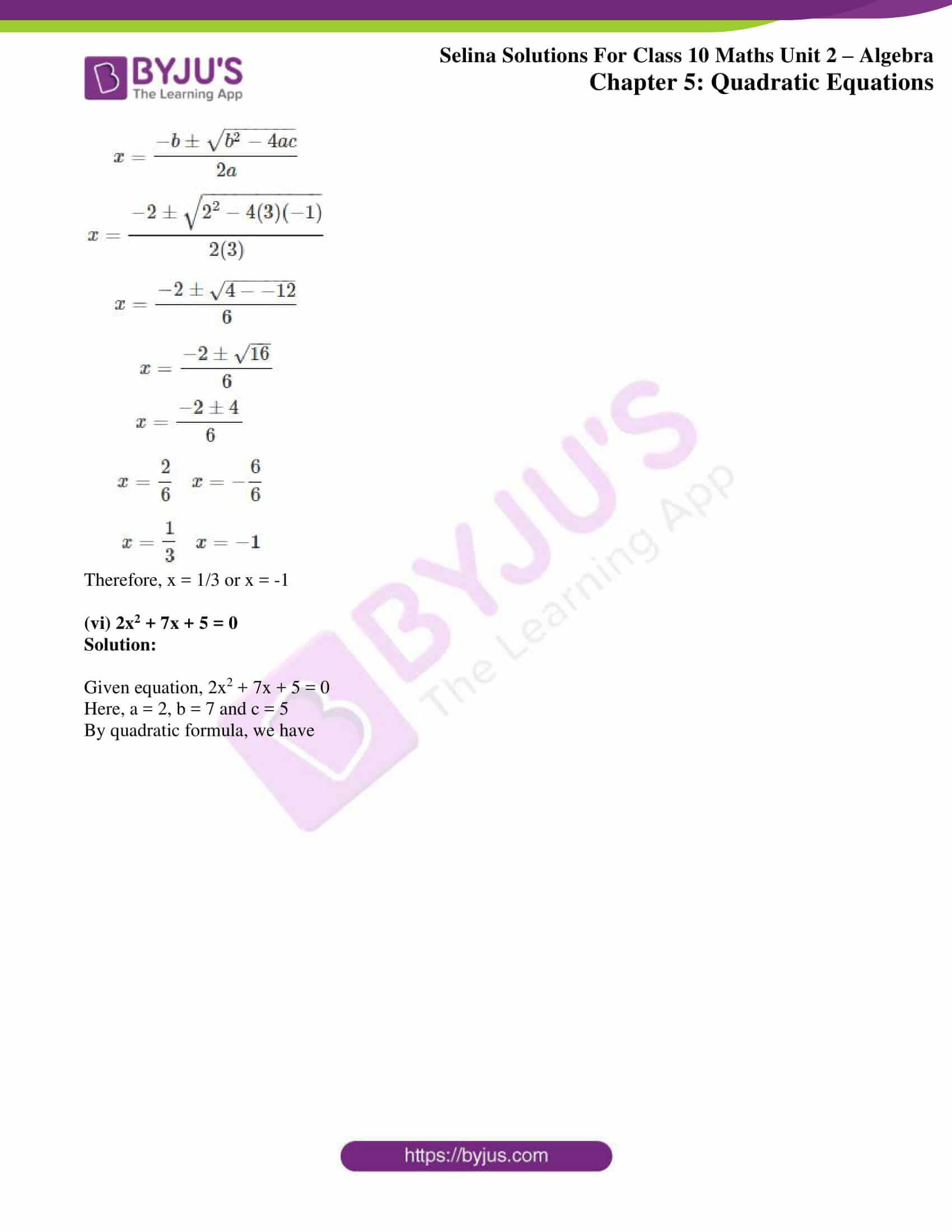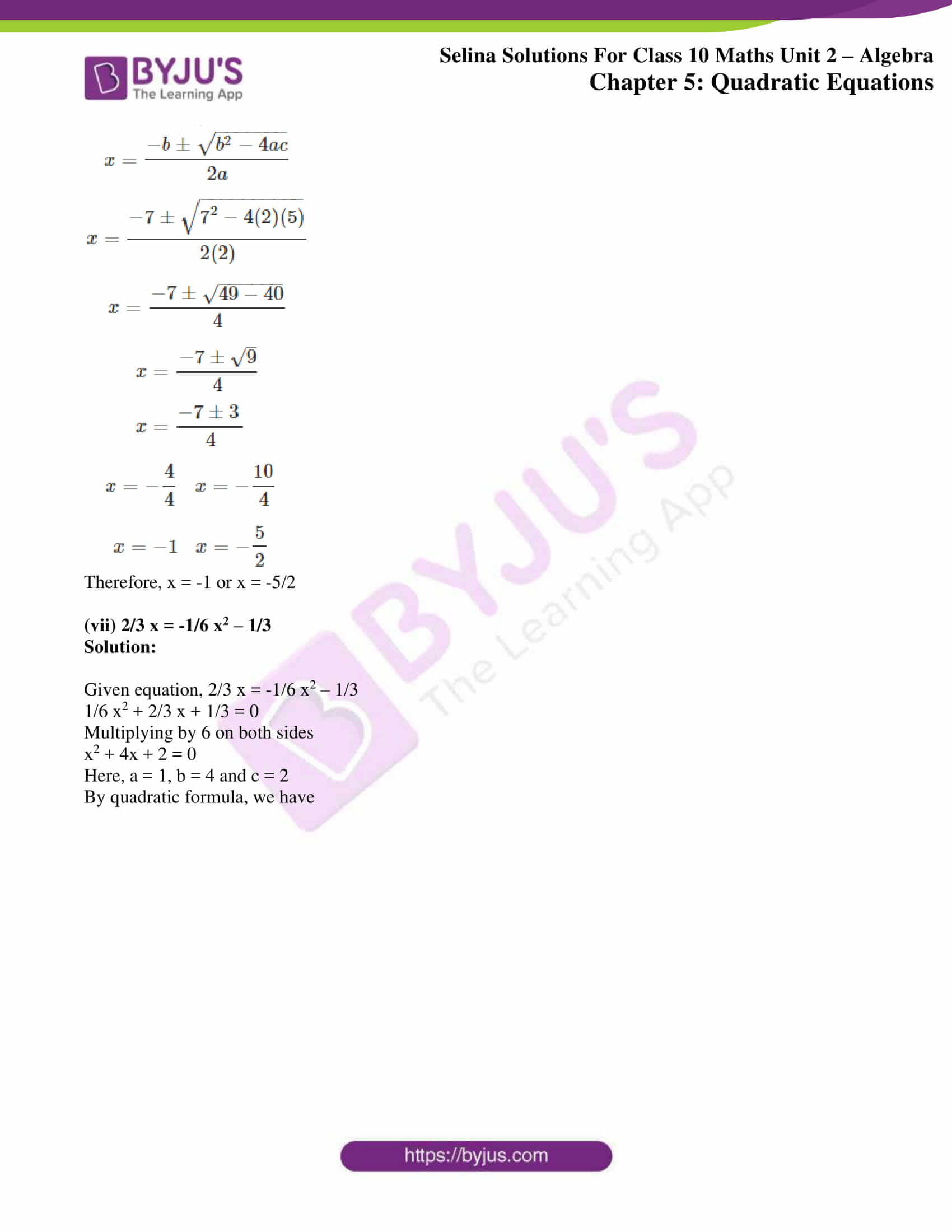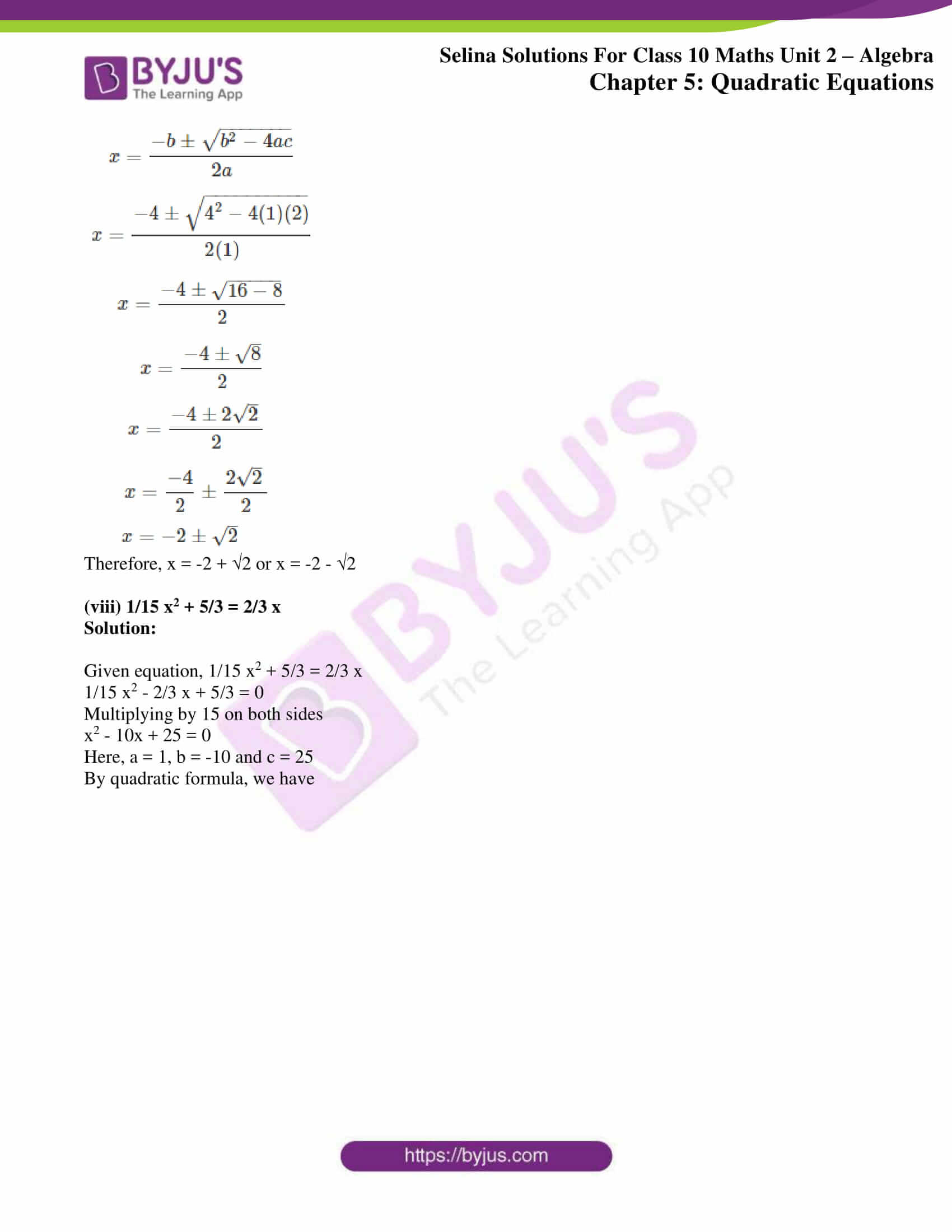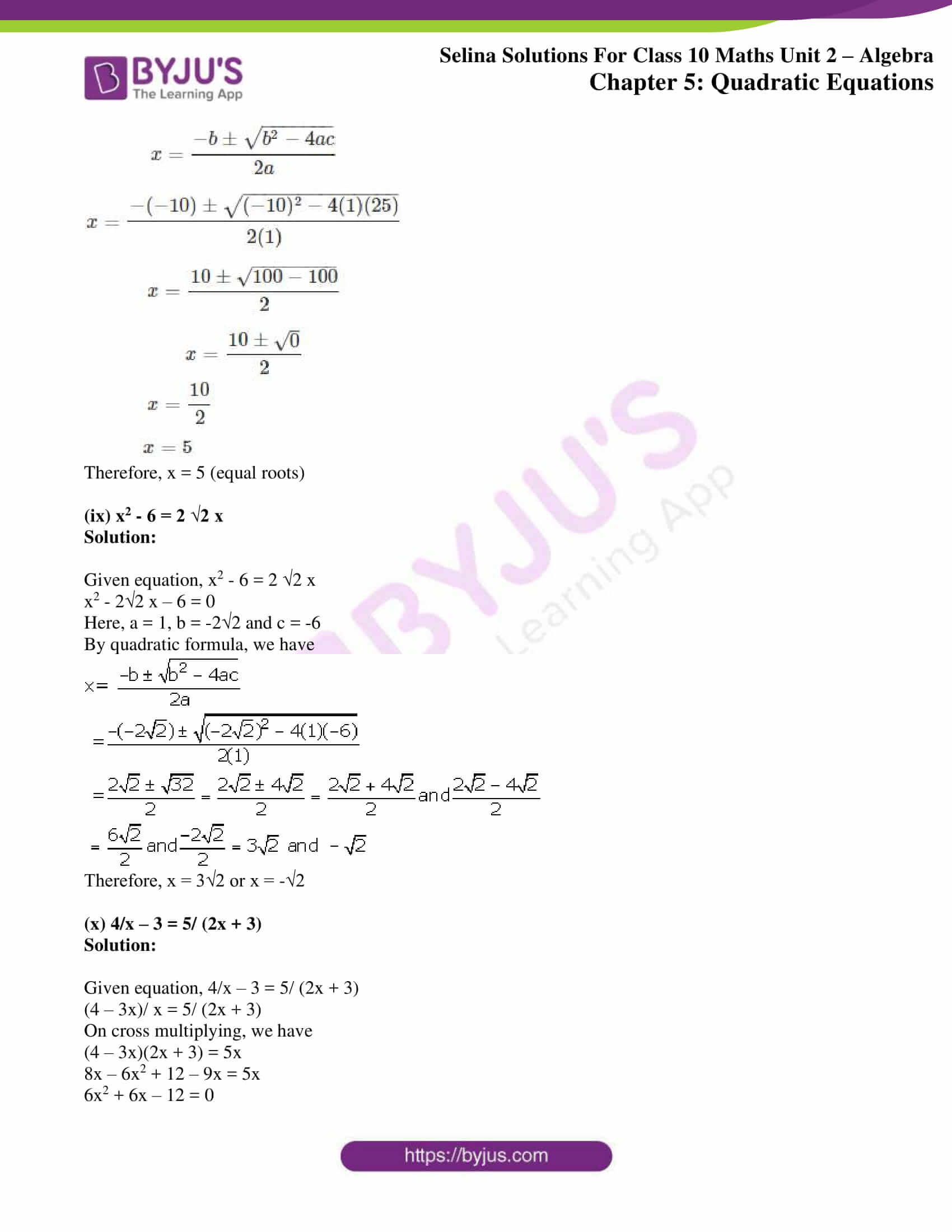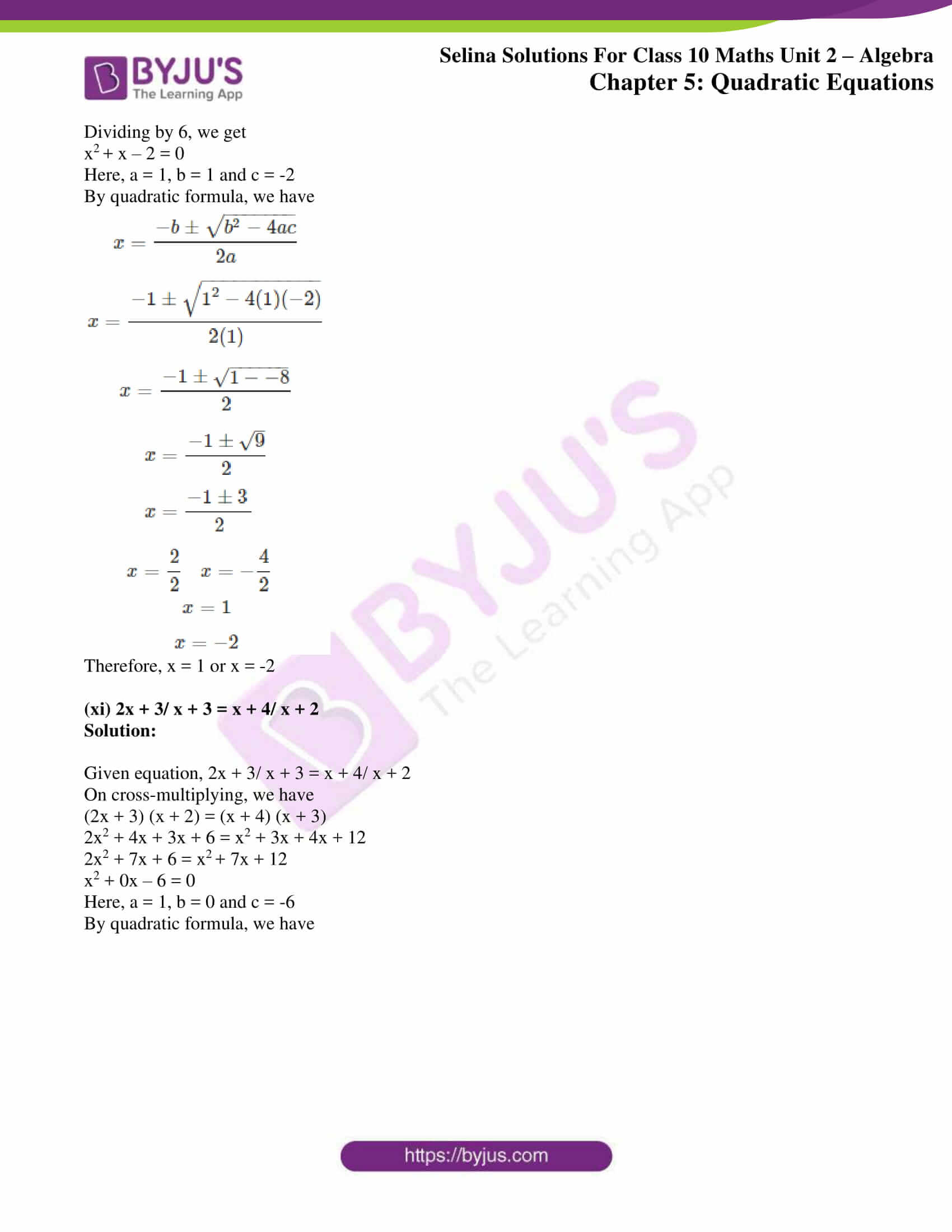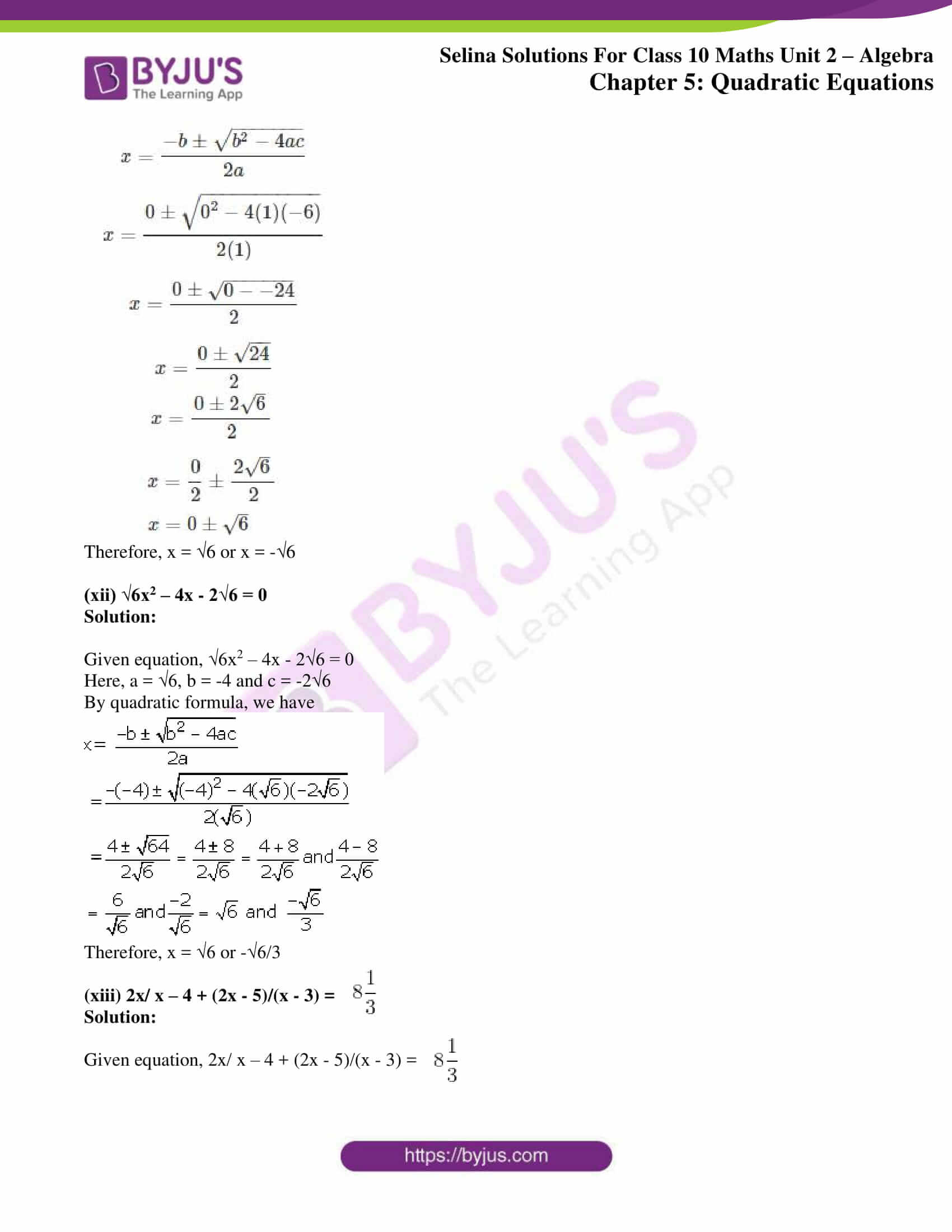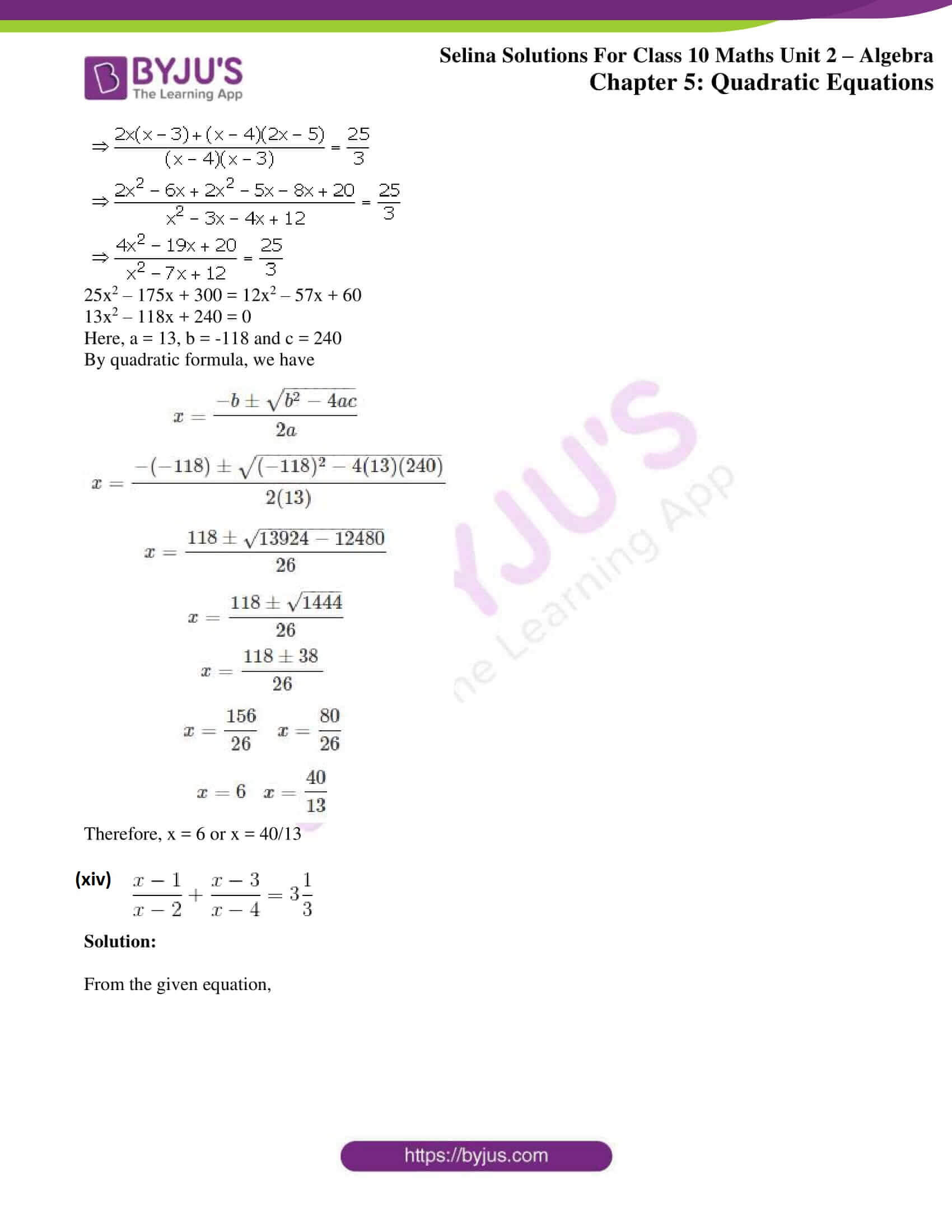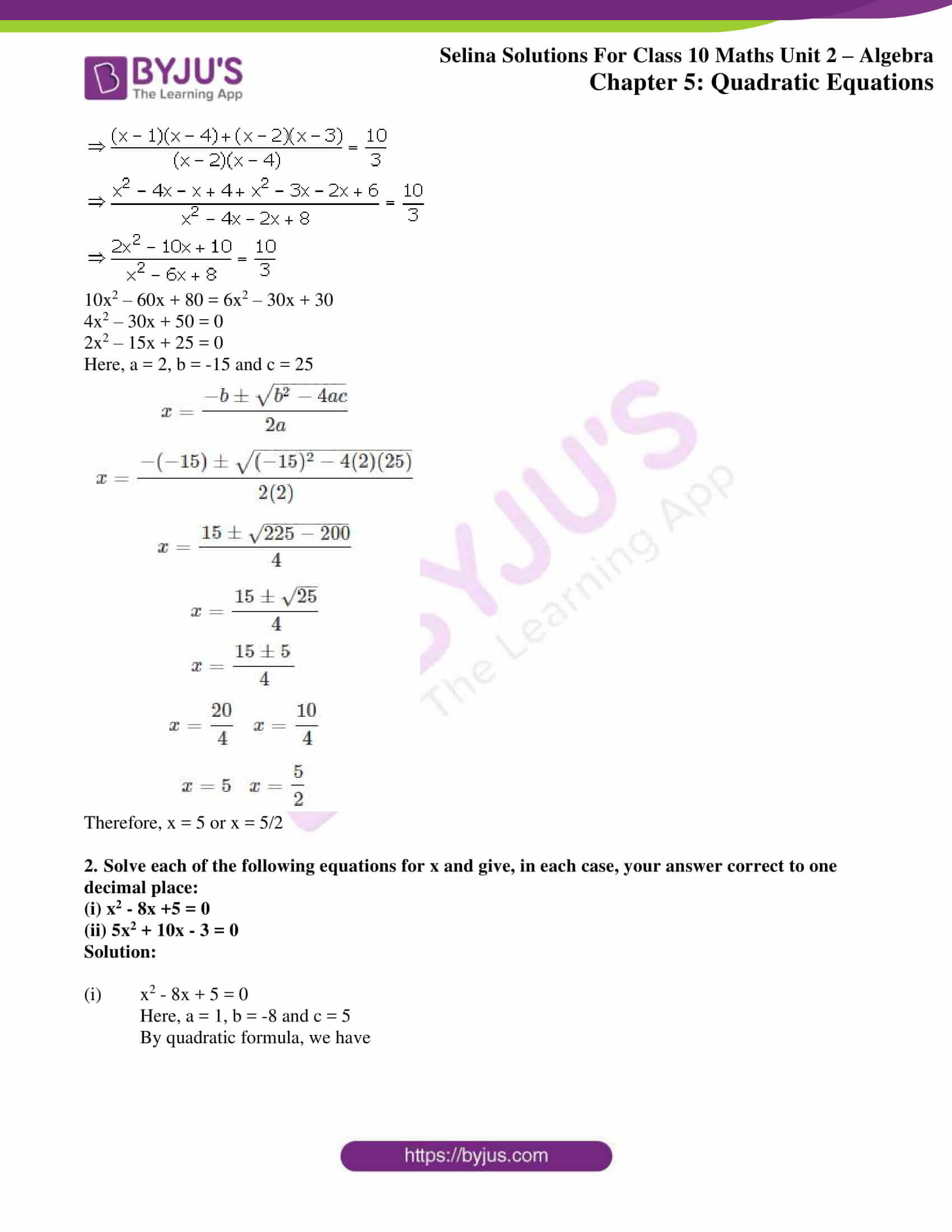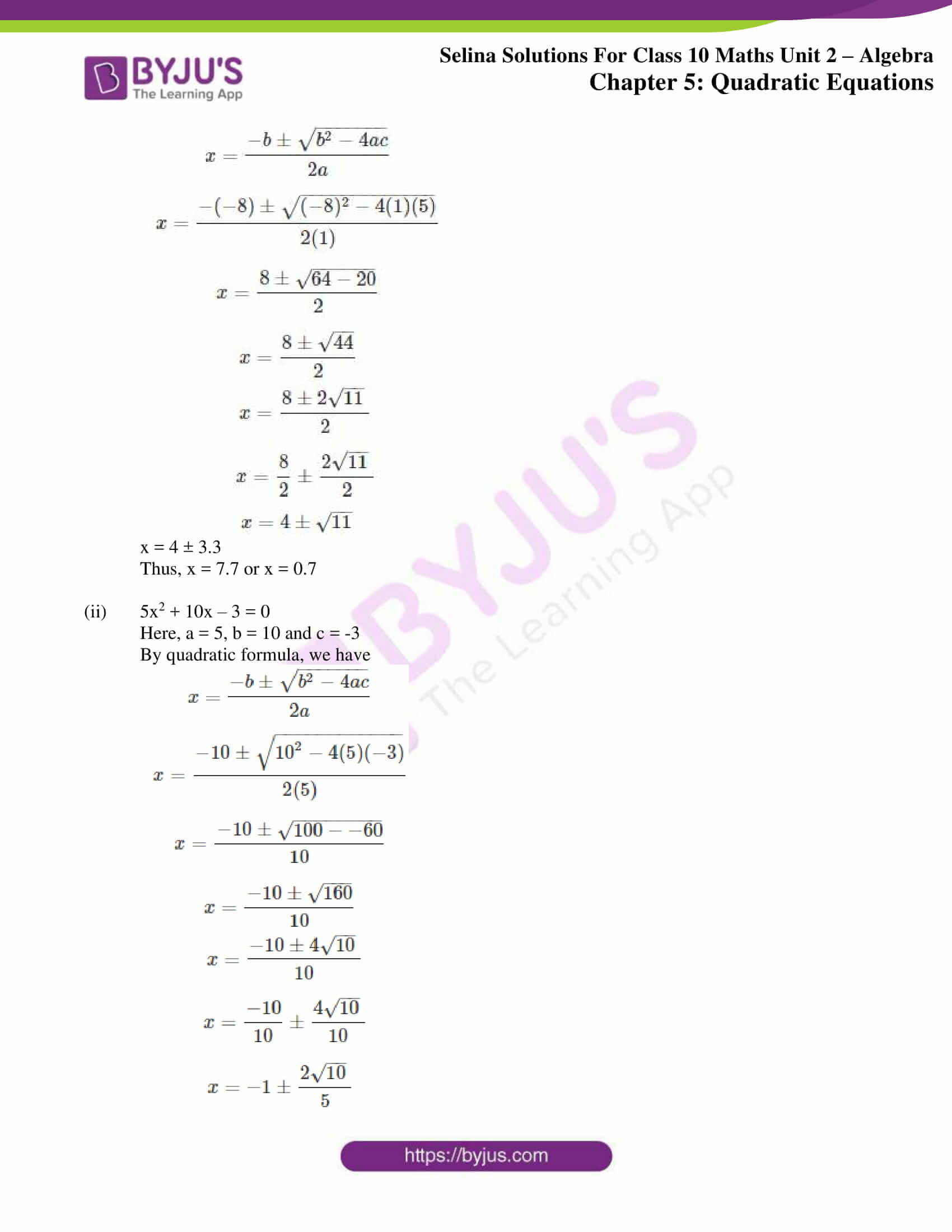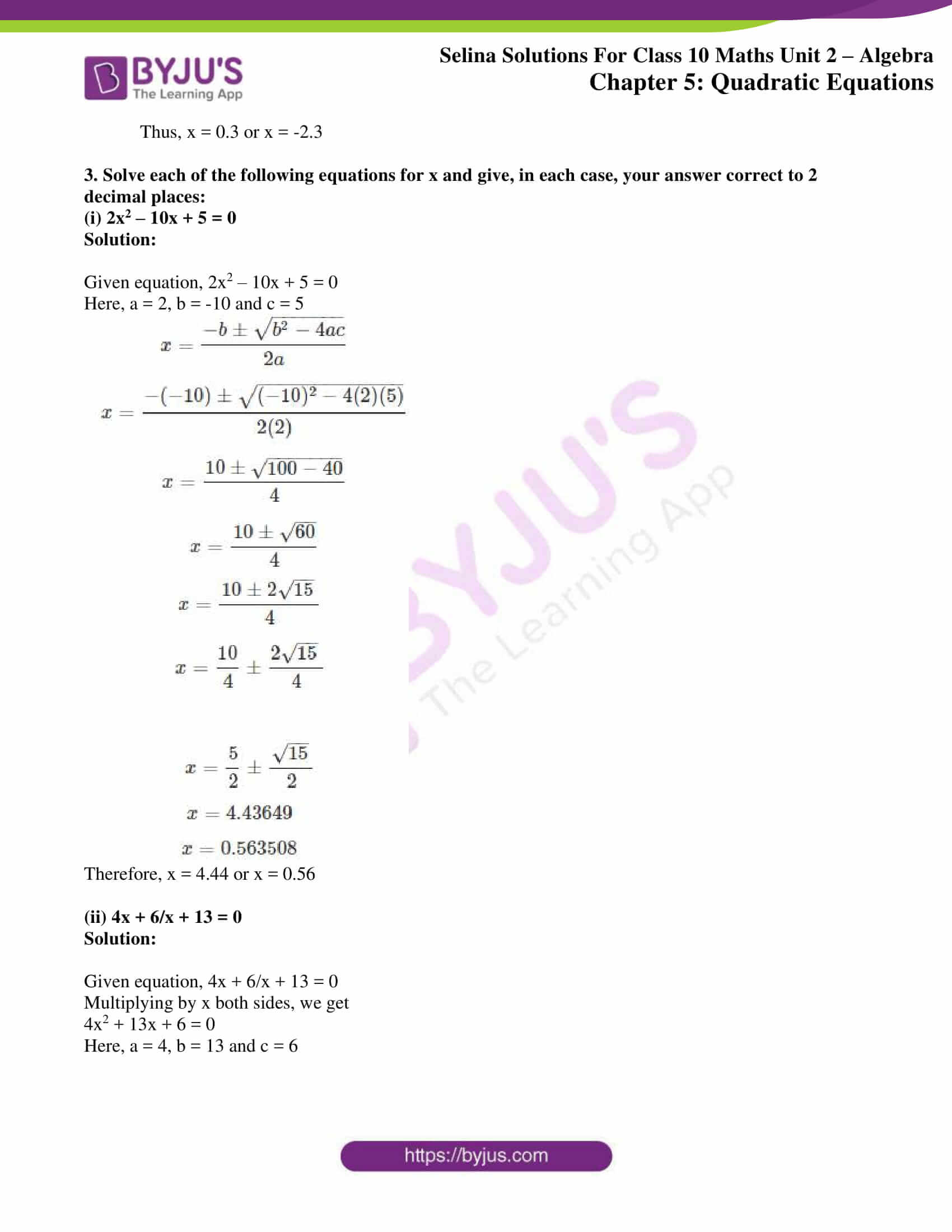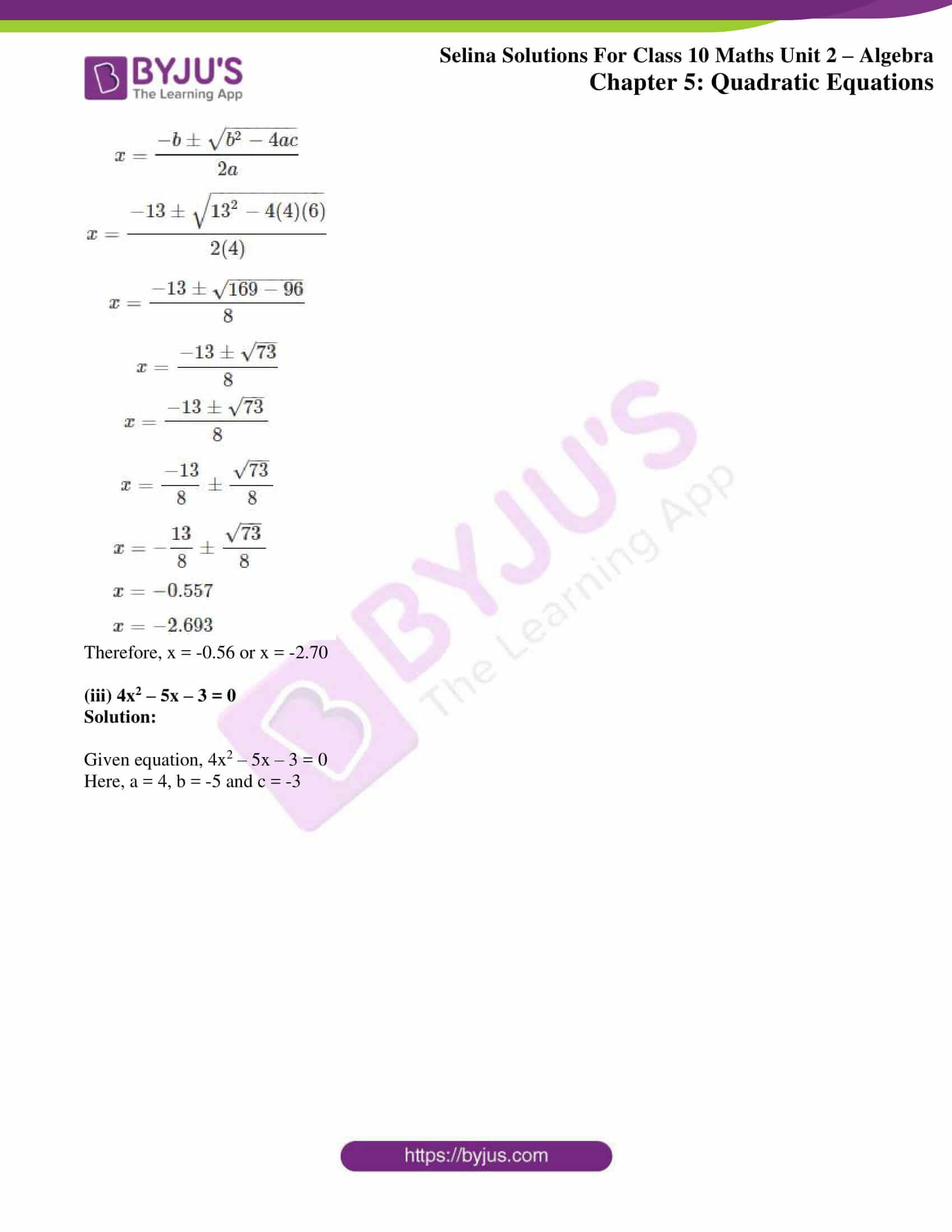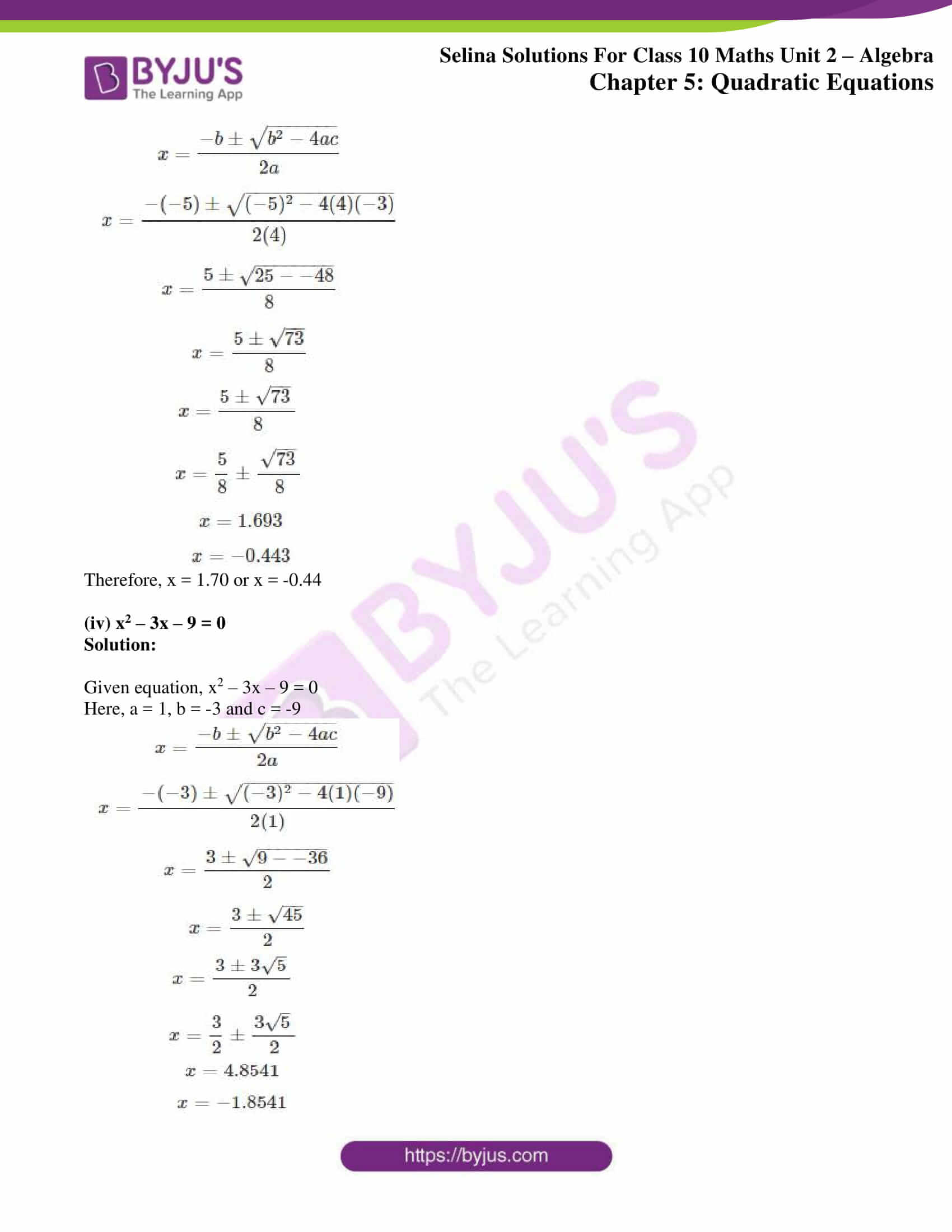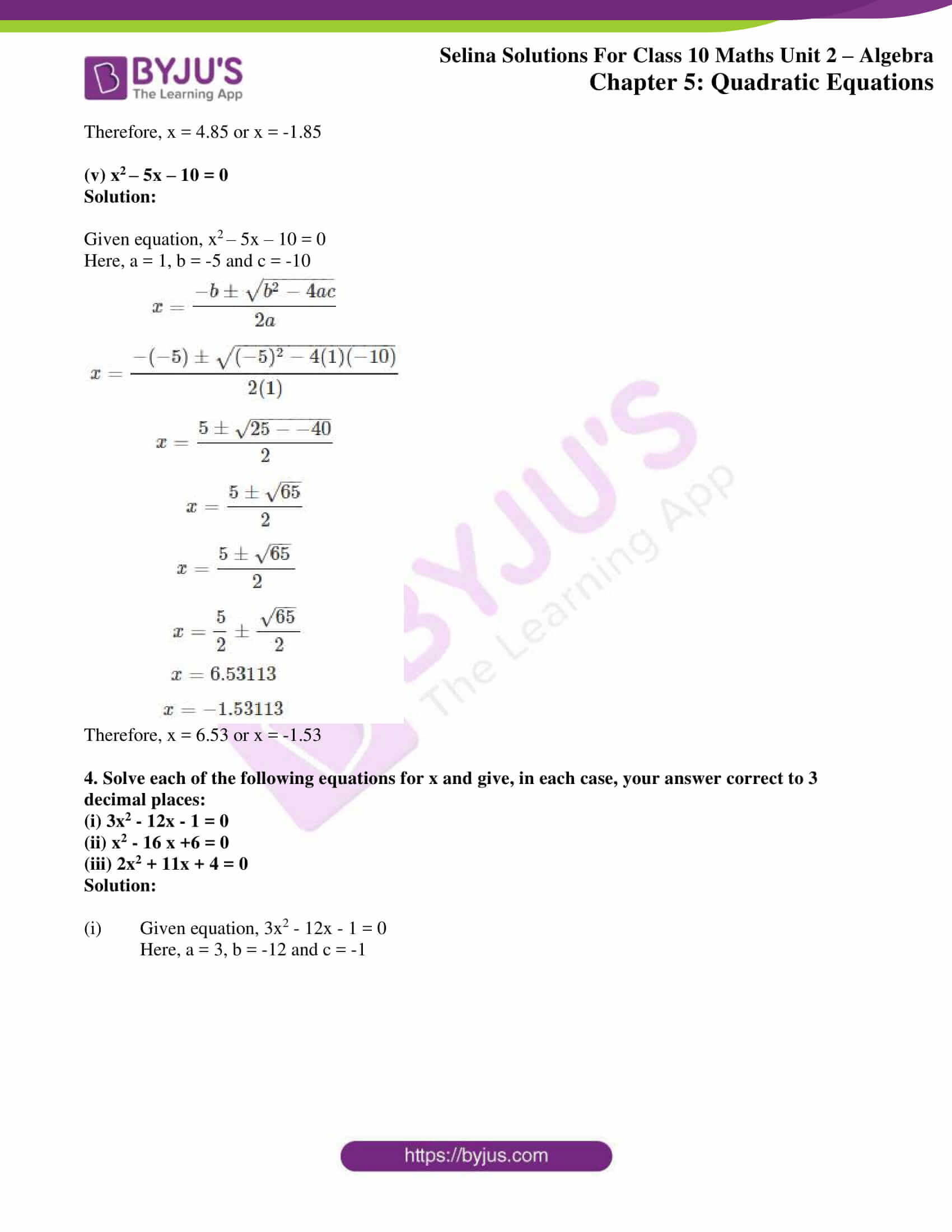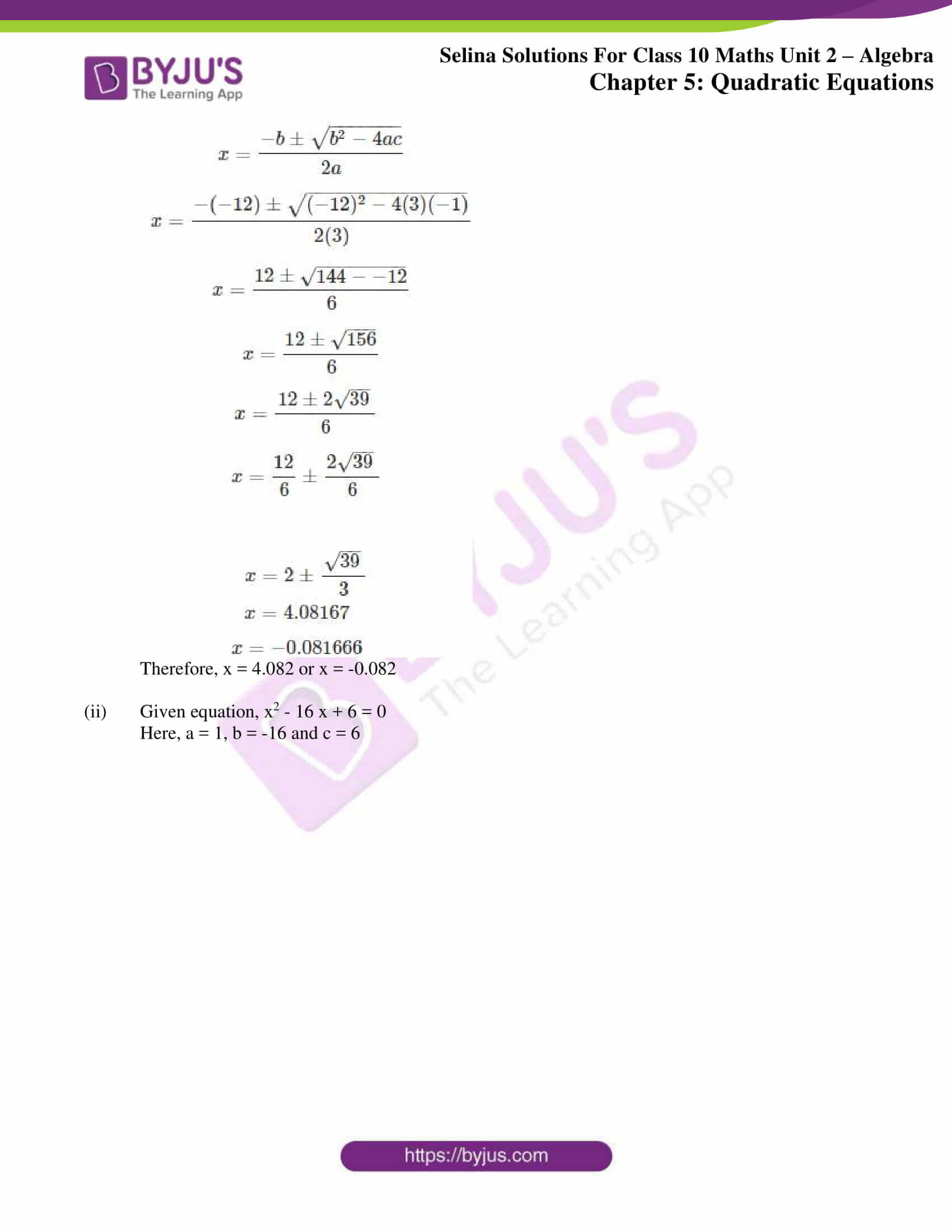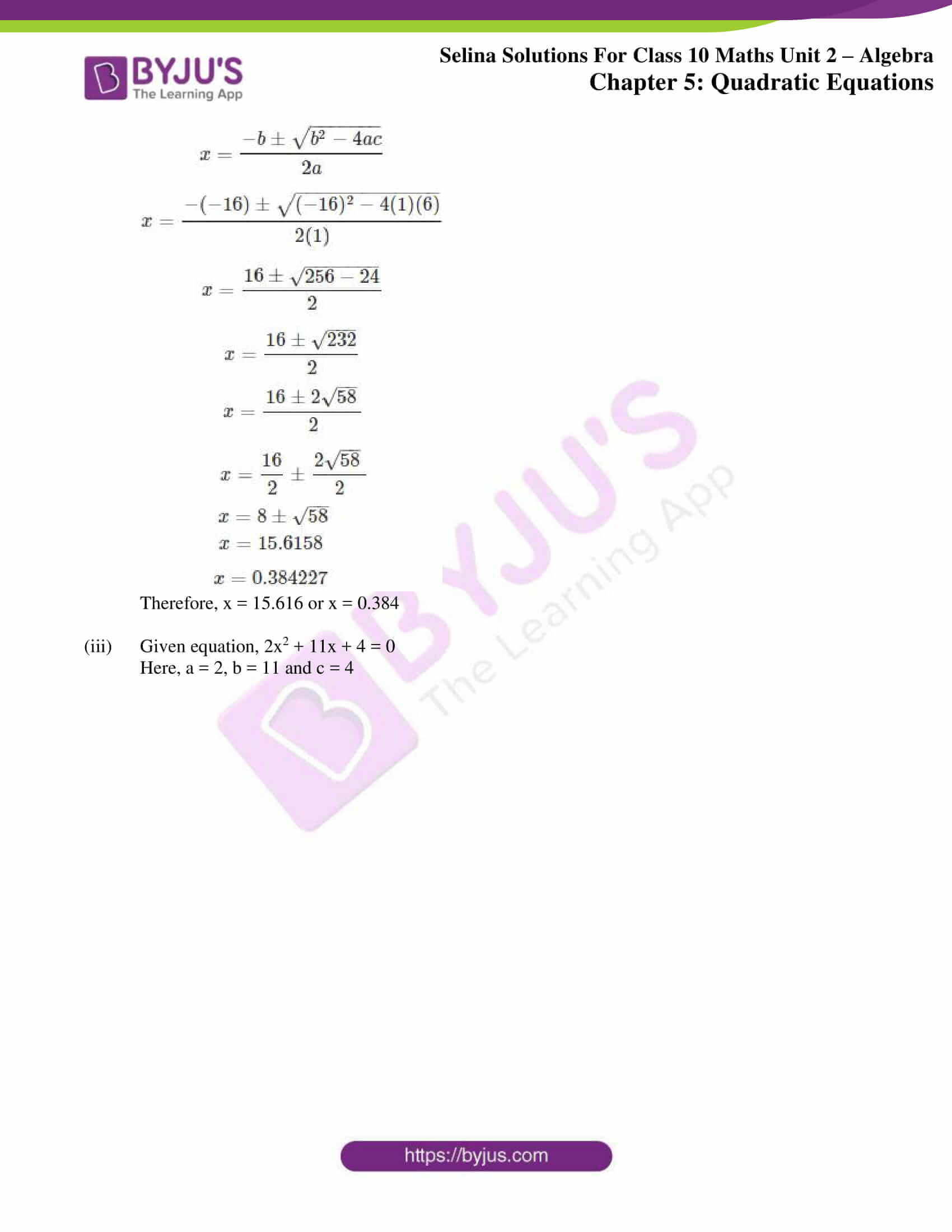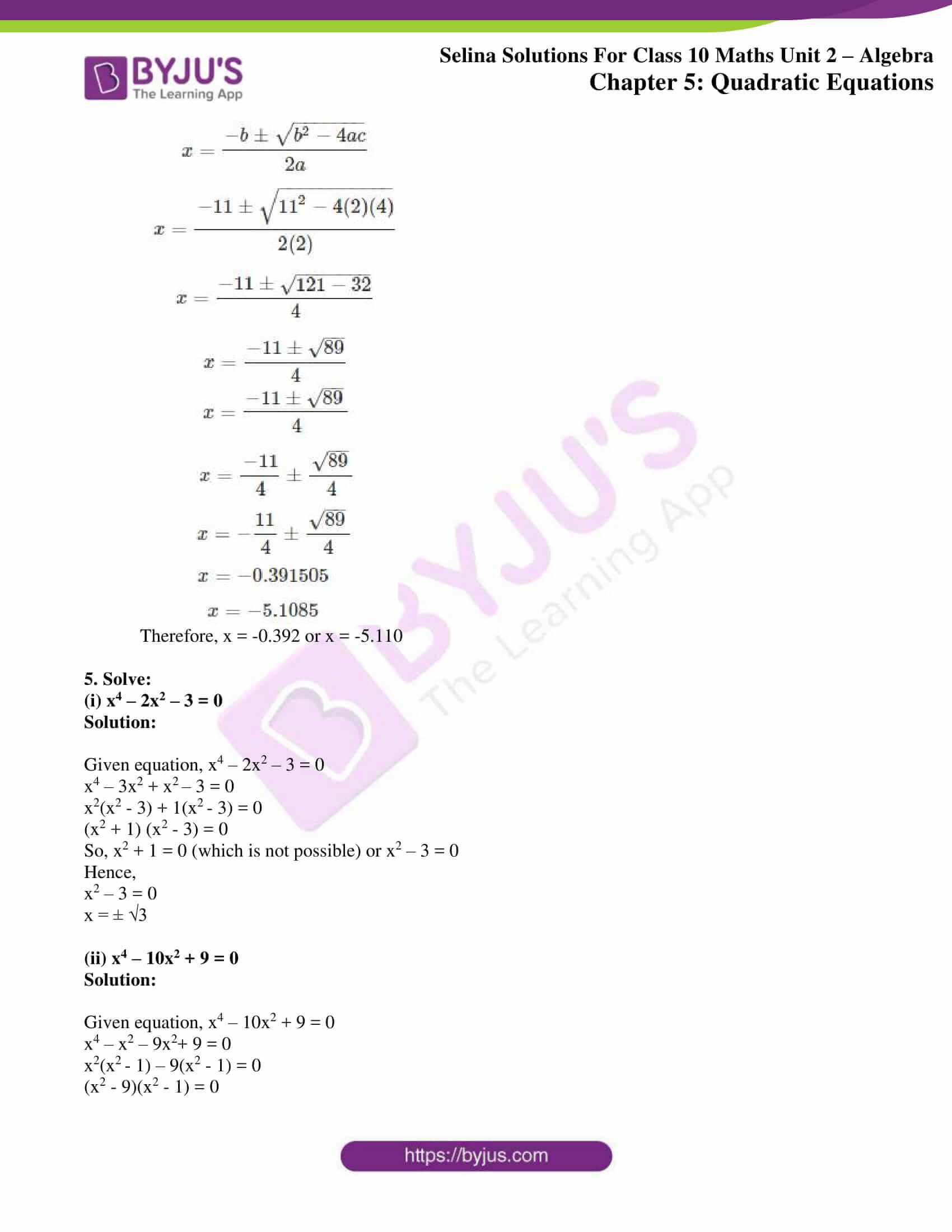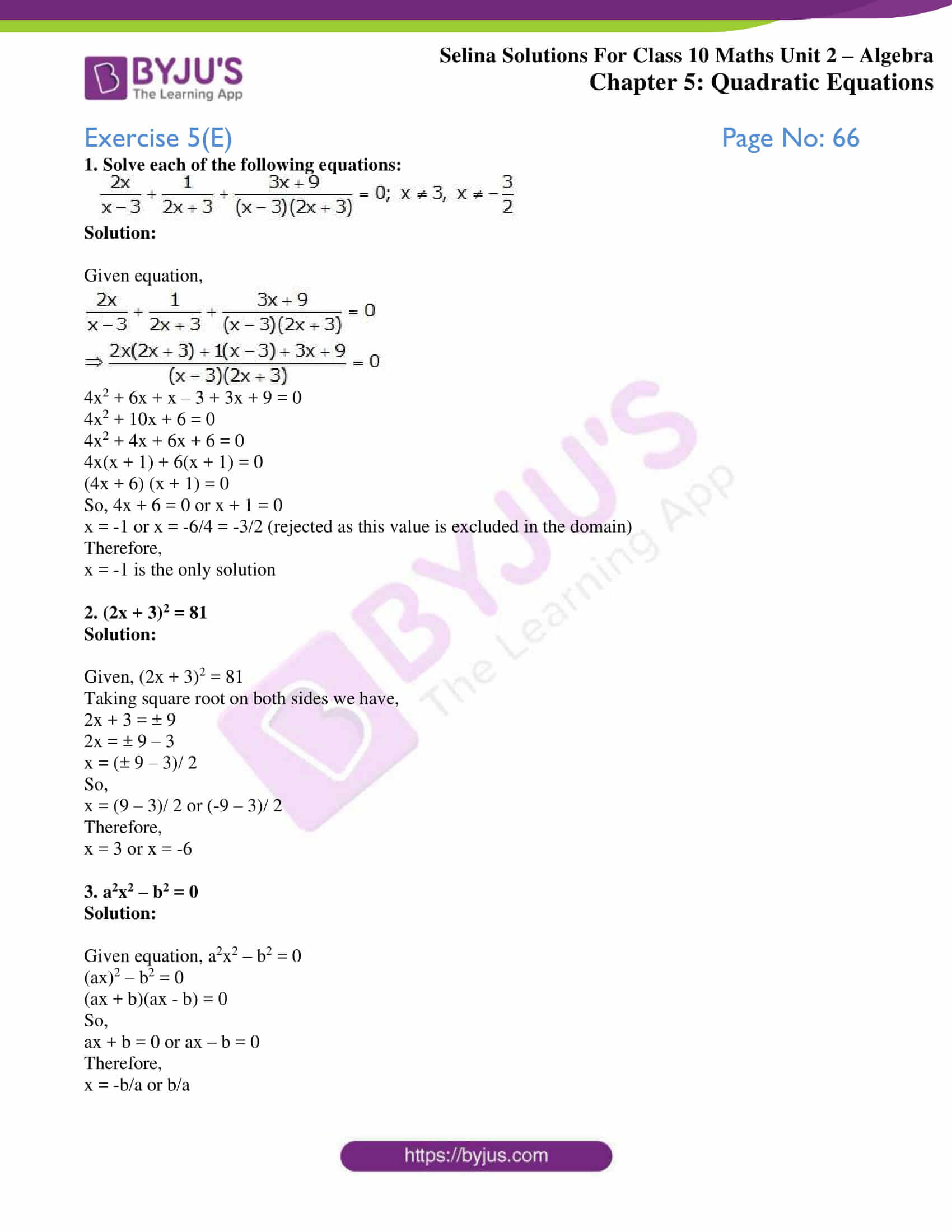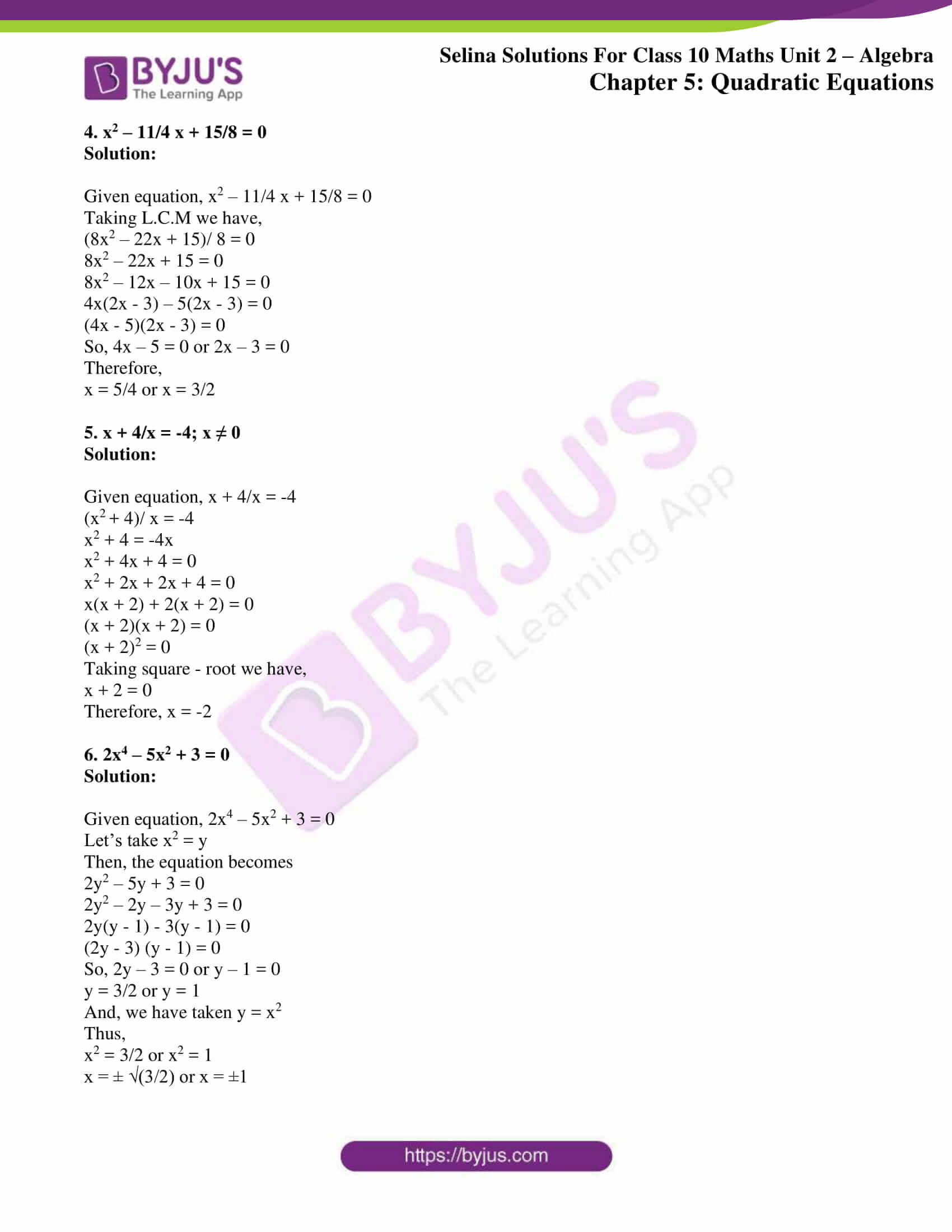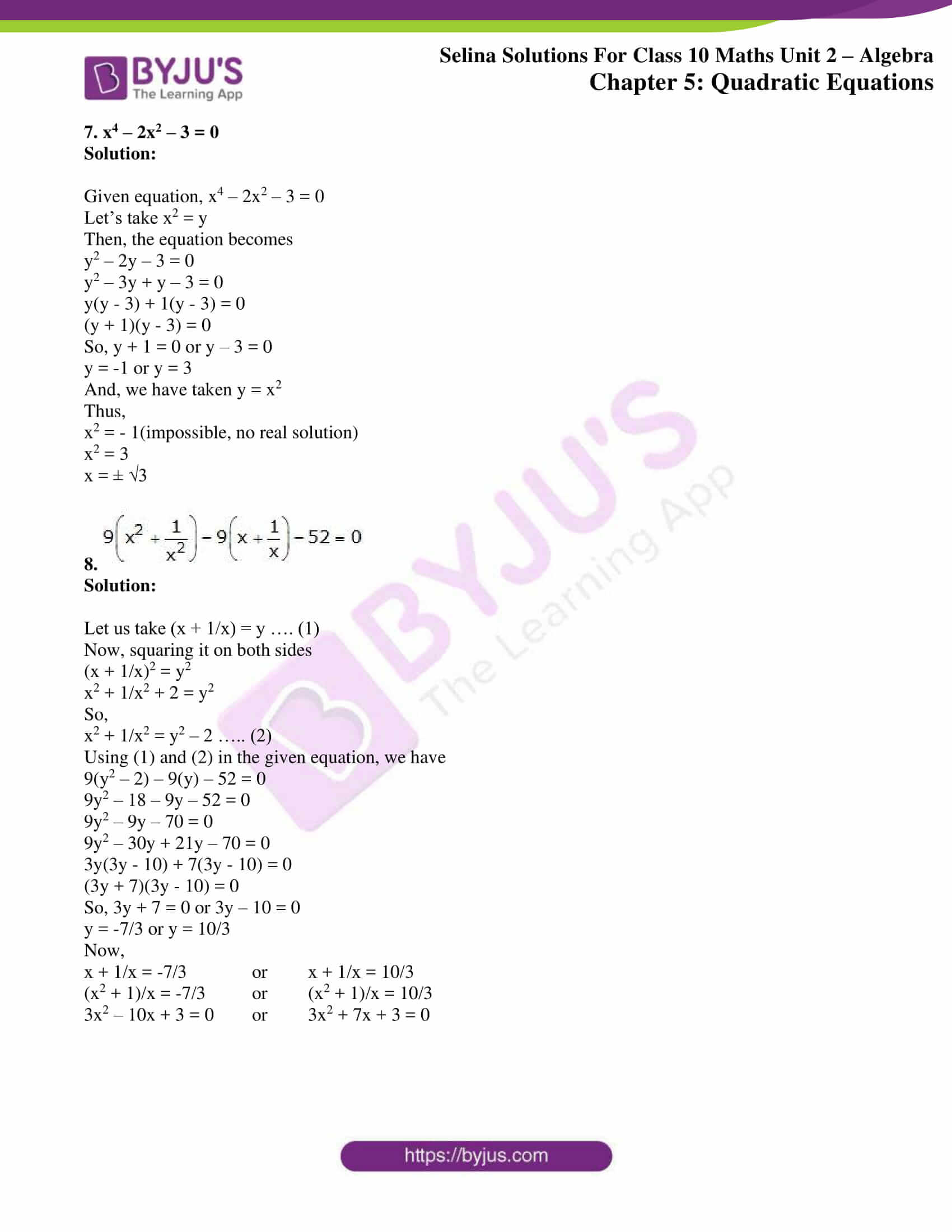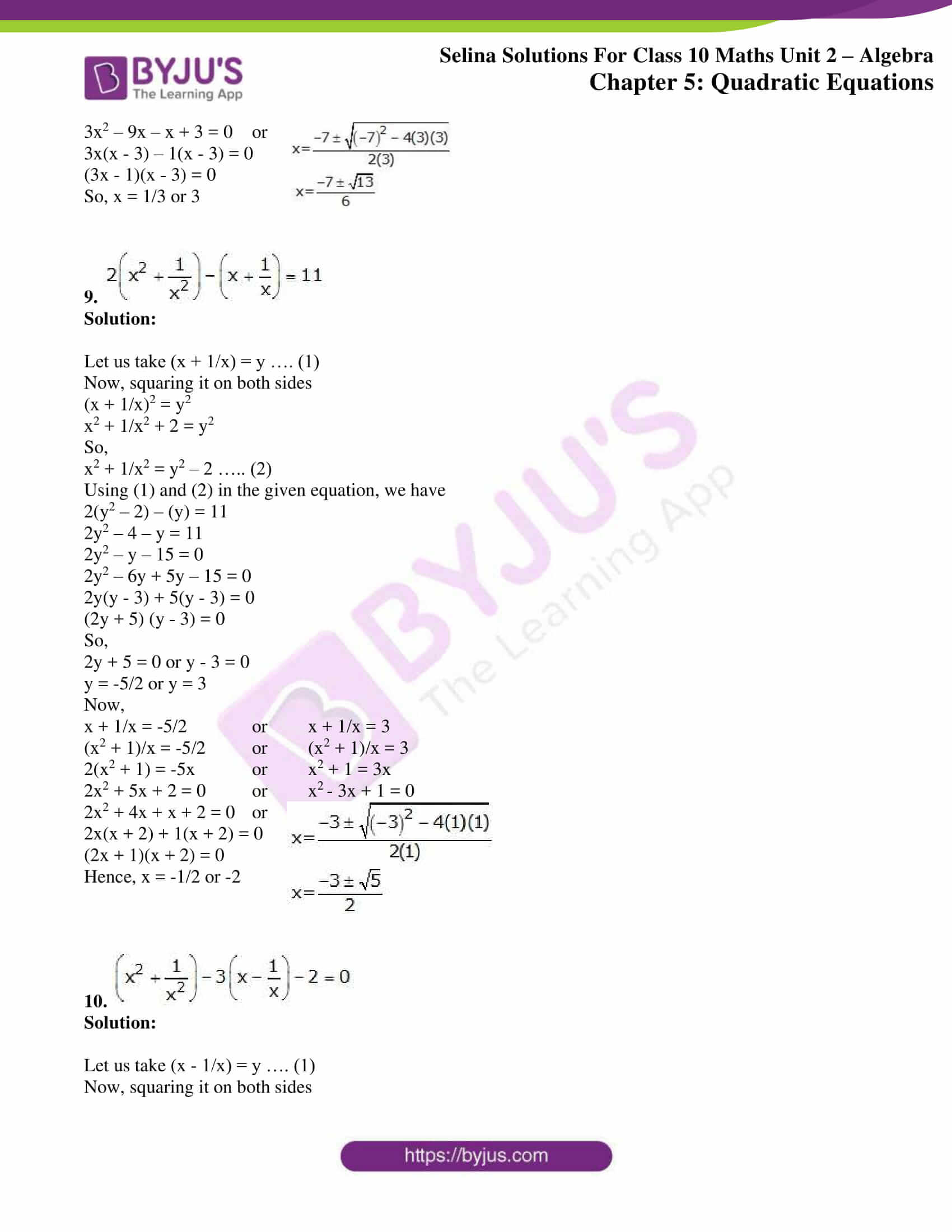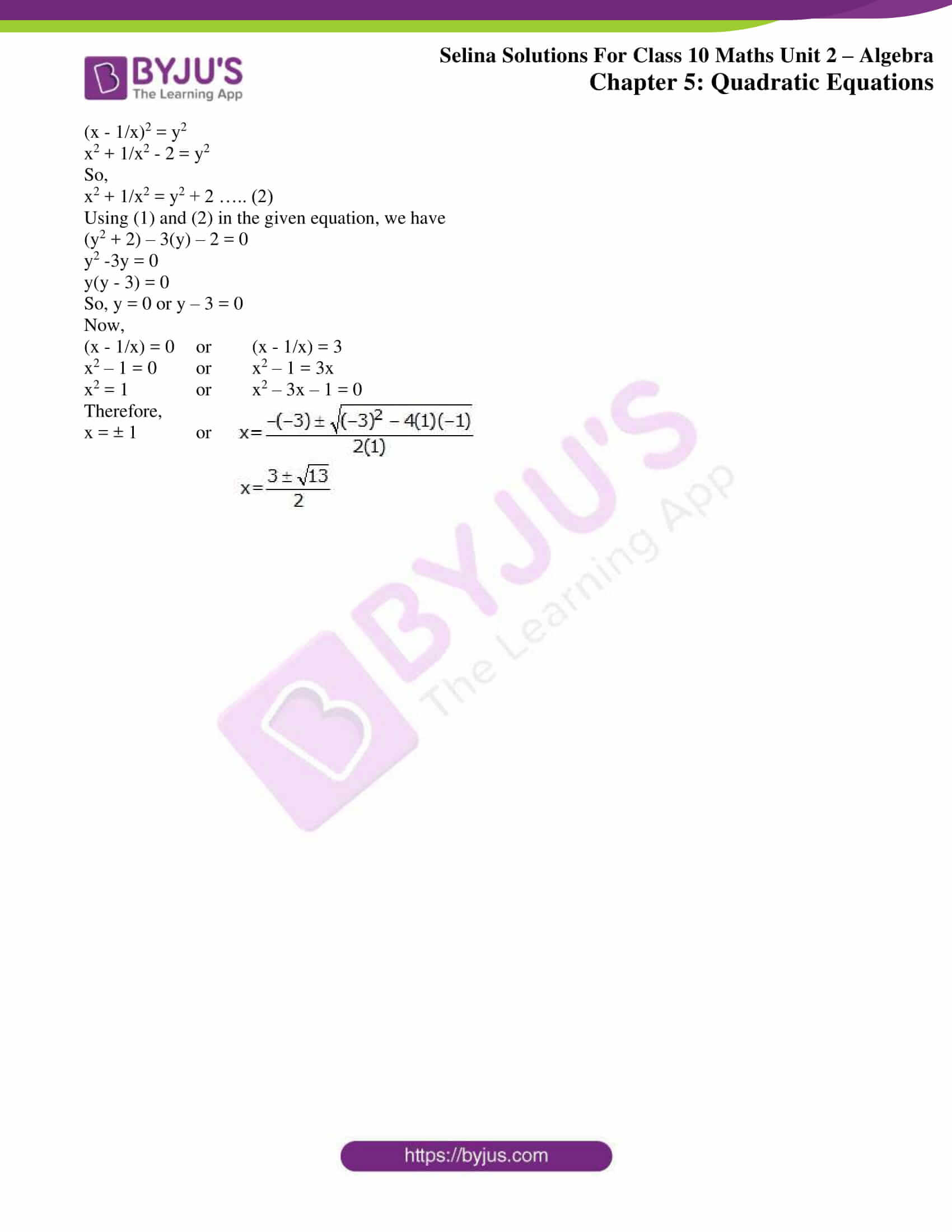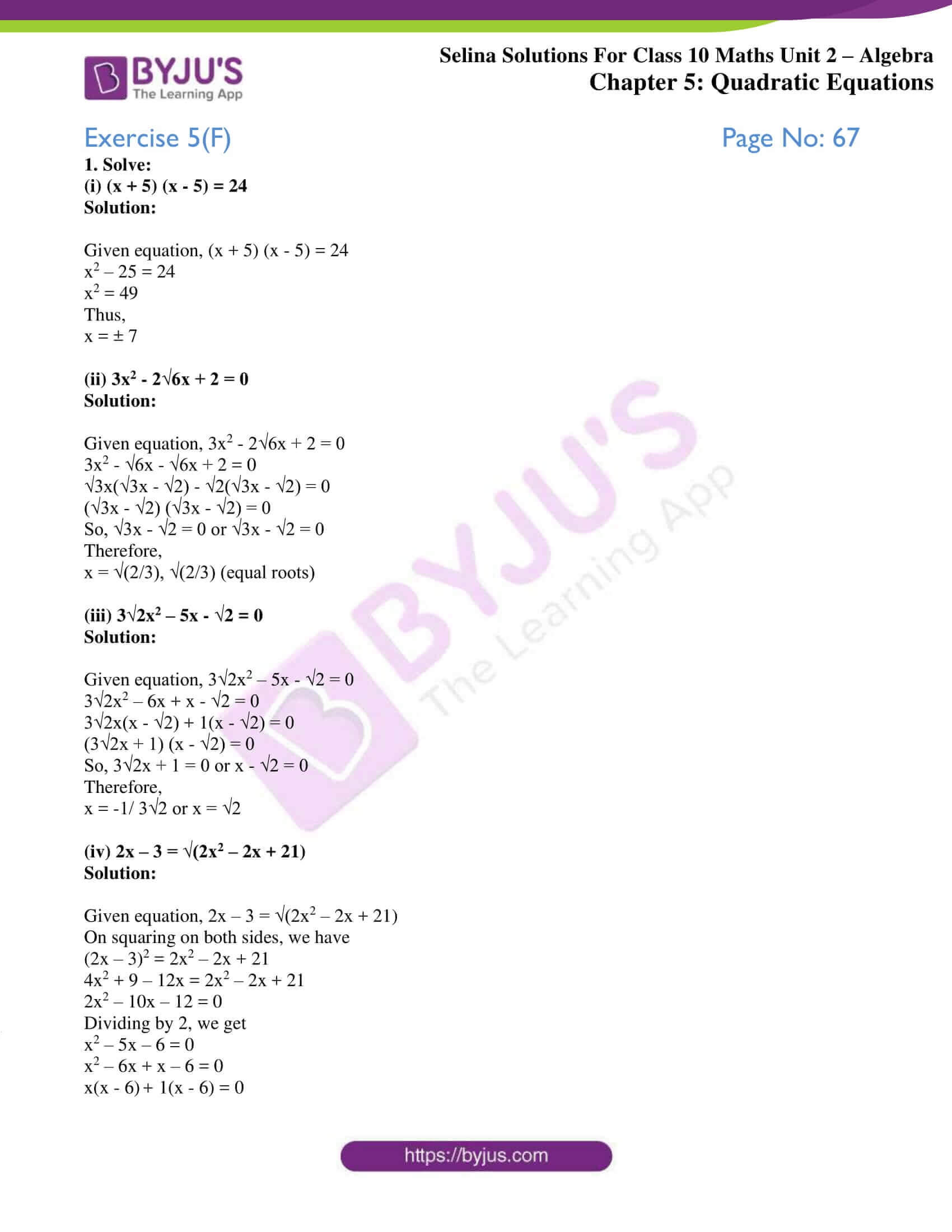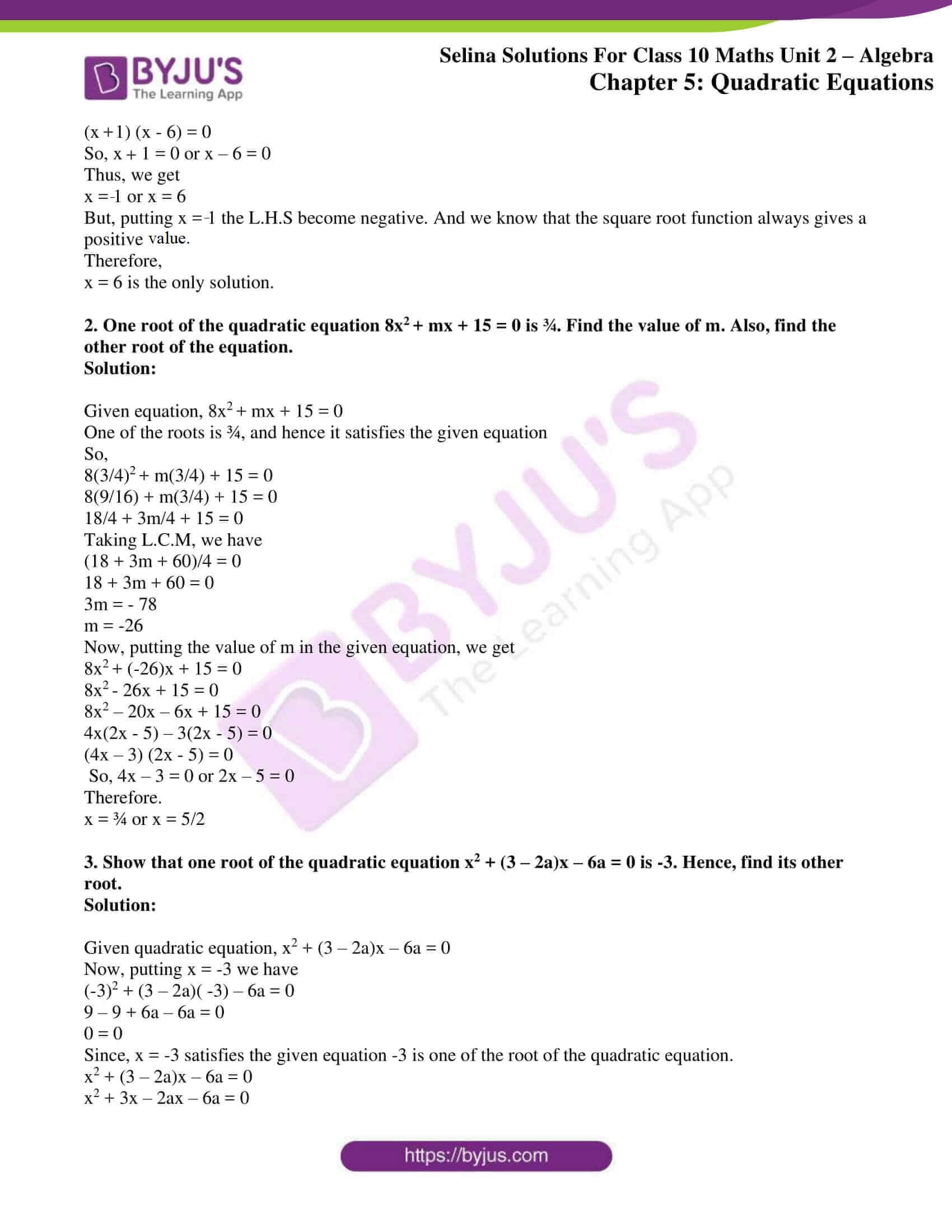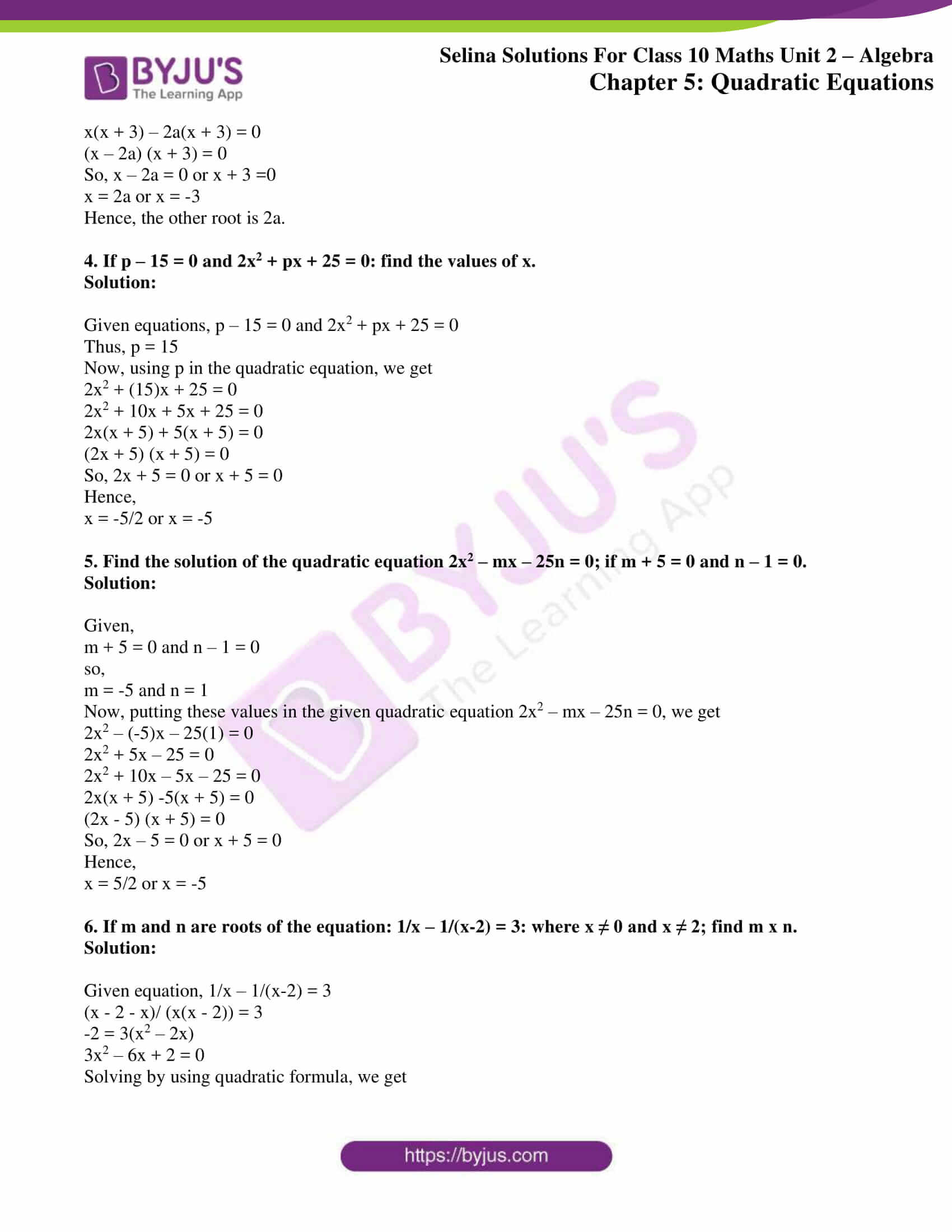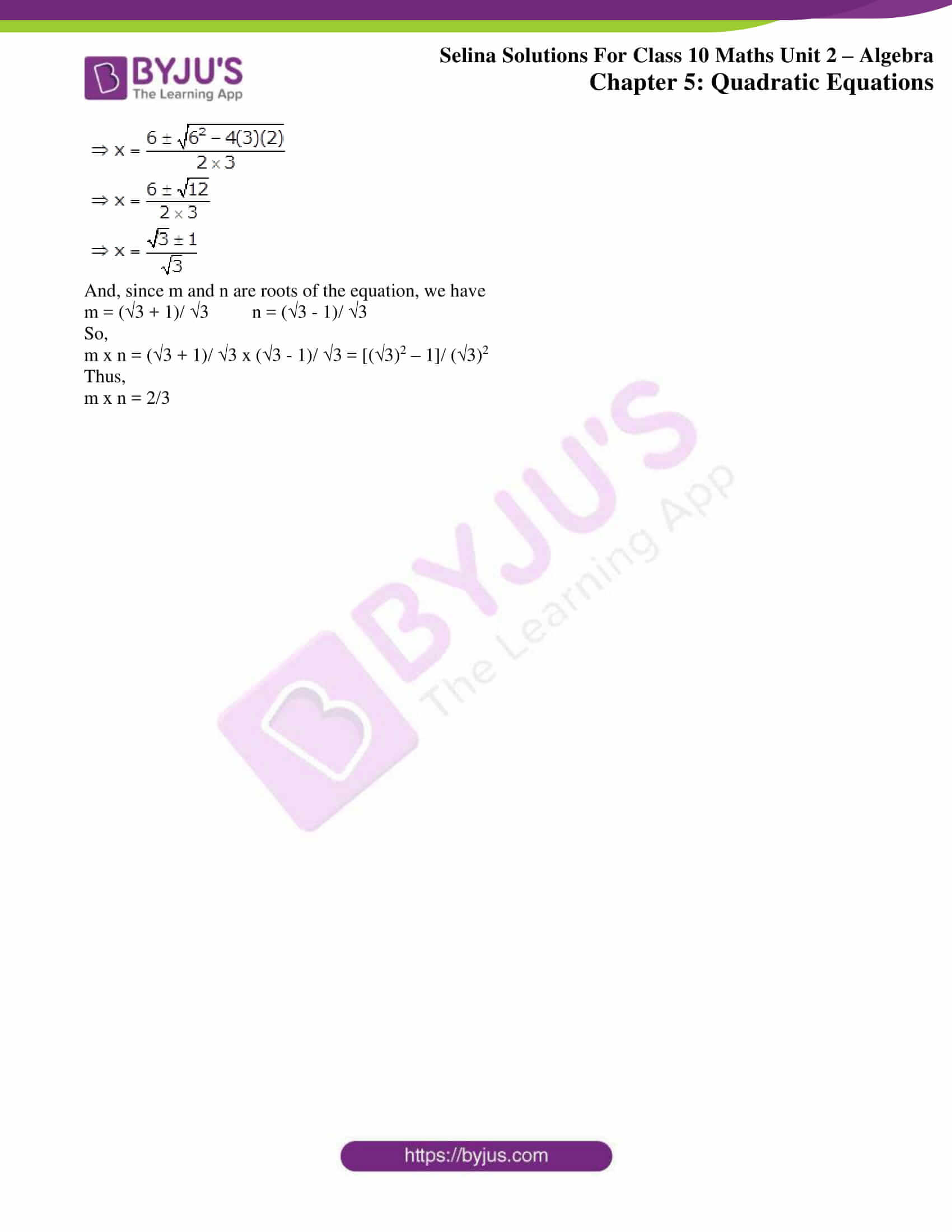### Exercises of Concise Selina Solutions Class 10 Maths Chapter 5 Quadratic Equations

Exercise 5(A) Solutions

Exercise 5(B) Solutions

Exercise 5(C) Solutions

Exercise 5(D) Solutions

Exercise 5(E) Solutions

Exercise 5(F) Solutions

## Access Selina Solutions Concise Maths Class 10 Chapter 5 Quadratic Equations

Exercise 5(A) Page No: 54

1. Find which of the following equations are quadratic:

(i) (3x – 1)2 = 5(x + 8)

(ii) 5x2 – 8x = -3(7 – 2x)

(iii) (x -4) (3x + 1) = (3x – 1) (x + 2)

(iv) x2 + 5x – 5 = (x – 3)2

(v) 7x3 – 2x2 + 10 = (2x – 5)2

(vi) (x – 1)2 + (x + 2)2 + 3(x + 1) = 0

Solution:

(i) (3x – 1)2 = 5(x + 8)

⇒ (9x2 – 6x + 1) = 5x + 40

⇒ 9x2 – 11x – 39 = 0; which is of the general form ax2 + bx + c = 0.

Thus, the given equation is a quadratic equation.

(ii) 5x2 – 8x = -3(7 – 2x)

⇒ 5x2 – 8x = 6x – 21

⇒ 5x2 – 14x + 21 = 0; which is of the general form ax2 + bx + c = 0.

Thus, the given equation is a quadratic equation.

(iii) (x – 4) (3x + 1) = (3x – 1) (x +2)

⇒ 3x2 + x – 12x – 4 = 3x2 + 6x – x – 2

⇒ 16x + 2 = 0; which is not of the general form ax2 + bx + c = 0. And it’s a linear equation.

Thus, the given equation is not a quadratic equation.

(iv) x2 + 5x – 5 = (x – 3)2

⇒ x2 + 5x – 5 = x2 – 6x + 9

⇒ 11x – 14 = 0; which is not of the general form ax2 + bx + c = 0. And it’s a linear equation.

Thus, the given equation is not a quadratic equation.

(v) 7x3 – 2x2 + 10 = (2x – 5)2

⇒ 7x3 – 2x2 + 10 = 4x2 – 20x + 25

⇒ 7x3 – 6x2 + 20x – 15 = 0; which is not of the general form ax2 + bx + c = 0. And it’s a cubic equation.

Thus, the given equation is not a quadratic equation.

(vi) (x – 1)2 + (x + 2)2 + 3(x +1) = 0

⇒ x2 – 2x + 1 + x2 + 4x + 4 + 3x + 3 = 0

⇒ 2x2 + 5x + 8 = 0; which is of the general form ax2 + bx + c = 0.

Thus, the given equation is a quadratic equation.

2. (i) Is x = 5 a solution of the quadratic equation x2 – 2x – 15 = 0?

Solution:

Given quadratic equation, x2 – 2x – 15 = 0

We know that, for x = 5 to be a solution of the given quadratic equation it should satisfy the equation.

Now, on substituting x = 5 in the given equation, we have

L.H.S = (5)2 – 2(5) – 15

= 25 – 10 – 15

= 0

= R.H.S

Therefore, x = 5 is a solution of the given quadratic equation x2 – 2x – 15 = 0

(ii) Is x = -3 a solution of the quadratic equation 2x2 – 7x + 9 = 0?

Solution:

Given quadratic equation, 2x2 – 7x + 9 = 0

We know that, for x = -3 to be solution of the given quadratic equation it should satisfy the equation.

Now, on substituting x = 5 in the given equation, we have

L.H.S = 2(-3)2 – 7(-3) + 9

= 18 + 21 + 9

= 48

≠ R.H.S

Therefore, x = -3 is not a solution of the given quadratic equation 2x2 – 7x + 9 = 0.

Exercise 5(B) Page No: 56

1. Without solving, comment upon the nature of roots of each of the following equations:

(i) 7x2 – 9x +2 = 0 (ii) 6x2 – 13x +4 = 0

(iii) 25x2 – 10x + 1= 0 (iv) x2 + 2√3x – 9 = 0

(v) x2 – ax – b2 = 0 (vi) 2x2 + 8x + 9 = 0

Solution:

(i) Given quadratic equation, 7x2 – 9x + 2 = 0

Here, a = 7, b = -9 and c = 2

So, the Discriminant (D) = b2 – 4ac

D = (-9)2 – 4(7)(2)

= 81 – 56

= 25

As D > 0, the roots of the equation is real and unequal.

(ii) Given quadratic equation, 6x2 – 13x + 4 = 0

Here, a = 6, b = -13 and c = 4

So, the Discriminant (D) = b2 – 4ac

D = (-13)2 – 4(6)(4)

= 169 – 96

= 73

As D > 0, the roots of the equation is real and unequal.

(iii) Given quadratic equation, 25x2 – 10x + 1 = 0

Here, a = 25, b = -10 and c = 1

So, the Discriminant (D) = b2 – 4ac

D = (-10)2 – 4(25)(1)

= 100 – 100

= 0

As D = 0, the roots of the equation is real and equal.

(iv) Given quadratic equation, x2 + 2√3x – 9 = 0

Here, a = 1, b = 2√3 and c = -9

So, the Discriminant (D) = b2 – 4ac

D = (2√3)2 – 4(1)(-9)

= 12 + 36

= 48

As D > 0, the roots of the equation is real and unequal.

(v) Given quadratic equation, x2 – ax – b2 = 0

Here, a = 1, b = -a and c = -b2

So, the Discriminant (D) = b2 – 4ac

D = (a)2 – 4(1)(-b2)

= a2 + 4b2

a2 + 4b2 is always positive value.

Thus D > 0, and the roots of the equation is real and unequal

(vi) Given quadratic equation, 2x2 + 8x + 9 = 0

Here, a = 2, b = 8 and c = 9

So, the Discriminant (D) = b2 – 4ac

D = (8)2 – 4(2)(9)

= 64 – 72

= -8

As D < 0, the equation has no roots.

2. Find the value of ‘p’, if the following quadratic equations has equal roots:

(i) 4x2 – (p – 2)x + 1 = 0

(ii) x2 + (p – 3)x + p = 0

Solution:

(i) 4x2 – (p – 2)x + 1 = 0

Here, a = 4, b = -(p – 2), c = 1

Given that the roots are equal,

So, Discriminant = 0 ⇒ b2– 4ac = 0

D = (-(p – 2))2 – 4(4)(1) = 0

⇒ p2 + 4 – 4p – 16 = 0

⇒ p2 – 4p – 12 = 0

⇒ p2 – 6p + 2p – 12 = 0

⇒ p(p – 6) + 2(p – 6) = 0

⇒ (p + 2)(p – 6) = 0

⇒ p + 2 = 0 or p – 6 = 0

Hence, p = -2 or p = 6

(ii) x2 + (p – 3)x + p = 0

Here, a = 1, b = (p – 3), c = p

Given that the roots are equal,

So, Discriminant = 0 ⇒ b2– 4ac = 0

D = (p – 3)2 – 4(1)(p) = 0

⇒ p2 + 9 – 6p – 4p = 0

⇒ p2– 10p + 9 = 0

⇒ p2-9p – p + 9 = 0

⇒ p(p – 9) – 1(p – 9) = 0

⇒ (p -9)(p – 1) = 0

⇒ p – 9 = 0 or p – 1 = 0

Hence, p = 9 or p = 1

Exercise 5(C) Page No: 59

Solve equations, number 1 to 20, given below, using factorization method:

1. x2 – 10x – 24 = 0

Solution:

Given equation, x2 – 10x – 24 = 0

x2 – 12x + 2x – 24 = 0

x(x – 12) + 2(x – 12) = 0

(x + 2)(x – 12) = 0

So, x + 2 = 0 or x – 12 = 0

Hence,

x = -2 or x = 12

2. x2 – 16 = 0

Solution:

Given equation, x2 – 16 = 0

x2 + 4x – 4x + 16 = 0

x(x + 4) -4(x + 4) = 0

(x – 4) (x + 4) = 0

So, (x – 4) = 0 or (x + 4) = 0

Hence,

x = 4 or x = -4

3. 2x2 – ½ x = 0

Solution:

Given equation, 2x2 – ½ x = 0

4x2 – x = 0

x(4x – 1) = 0

So, either x = 0 or 4x – 1 = 0

Hence,

x = 0 or x = ¼

4. x(x – 5) = 24

Solution:

Given equation, x(x – 5) = 24

x2 – 5x = 24

x2 – 5x – 24 = 0

x2 – 8x + 3x – 24 = 0

x(x – 8) + 3(x – 8) = 0

(x + 3)(x – 8) = 0

So, x + 3 = 0 or x – 8 = 0

Hence,

x = -3 or x = 8

5. 9/2 x = 5 + x2

Solution:

Given equation, 9/2 x = 5 + x2

On multiplying by 2 both sides, we have

9x = 2(5 + x2)

9x = 10 + 2x2

2x2 – 9x + 10 = 0

2x2 – 4x – 5x + 10 = 0

2x(x – 2) – 5(x – 2) = 0

(2x – 5)(x -2) = 0

So, 2x – 5 = 0 or x – 2 = 0

Hence,

x = 5/2 or x = 2

6. 6/x = 1 + x

Solution:

Given equation, 6/x = 1 + x

On multiplying by x both sides, we have

6 = x(1 + x)

6 = x + x2

x2 + x – 6 = 0

x2 + 3x – 2x – 6 = 0

x(x + 3) – 2(x + 3) = 0

(x – 2) (x + 3) = 0

So, x – 2 = 0 or x + 3 = 0

Hence,

x = 2 or x = -3

7. x = (3x + 1)/ 4x

Solution:

Given equation, x = (3x + 1)/ 4x

On multiplying by 4x both sides, we have

4x(x) = 3x + 1

4x2 = 3x + 1

4x2 – 3x – 1 = 0

4x2 – 4x + x – 1 = 0

4x(x – 1) + 1(x – 1) = 0

(4x + 1) (x – 1) = 0

So, 4x + 1 = 0 or x – 1 = 0

Hence,

x = -1/4 or x = 1

8. x + 1/x = 2.5

Solution:

Given equation, x + 1/x = 2.5

x + 1/x = 5/2

Taking LCM on L.H.S, we have

(x2 + 1)/ x = 5/2

2(x2 + 1) = 5x

2x2 + 2 = 5x

2x2 – 5x + 2 = 0

2x2 – 4x – x + 2 = 0

2x(x – 2) -1(x – 2) = 0

(2x – 1)(x – 2) = 0

So, 2x – 1 = 0 or x – 2 = 0

Hence,

x = ½ or x = 2

9. (2x – 3)2 = 49

Solution:

Given equation, (2x – 3)2 = 49

Expanding the L.H.S, we have

4x2 – 12x + 9 = 49

4x2 – 12x – 40 = 0

Dividing by 4 on both side

x2 – 3x – 10 = 0

x2 – 5x + 2x – 10 = 0

x(x – 5) + 2(x – 5) = 0

(x + 2) (x – 5) = 0

So, x + 2 = 0 or x – 5 = 0

Hence,

x = -2 or 5

10. 2(x2 – 6) = 3(x – 4)

Solution:

Given equation, 2(x2 – 6) = 3(x – 4)

2x2 – 12 = 3x – 12

2x2 = 3x

x(2x – 3) = 0

So, x = 0 or (2x – 3) = 0

Hence,

x = 0 or x = 3/2

11. (x + 1) (2x + 8) = (x + 7) (x + 3)

Solution:

Given equation, (x + 1) (2x + 8) = (x + 7) (x + 3)

2x2 + 2x + 8x + 8 = x2 + 7x + 3x + 21

2x2 + 10x + 8 = x2 + 10x + 21

x2 = 21 – 8

x2 – 13 = 0

(x – √13) (x + √13) = 0

So, x – √13 = 0 or x + √13 = 0

Hence,

x = – √13 or x = √13

12. x2 – (a + b)x + ab = 0

Solution:

Given equation, x2 – (a + b)x + ab = 0

x2 – ax – bx + ab = 0

x(x – a) – b(x – a) = 0

(x – b) (x – a) = 0

So, x – b = 0 or x – a = 0

Hence,

x = b or x = a

13. (x + 3)2 – 4(x + 3) – 5 = 0

Solution:

Given equation, (x + 3)2 – 4(x + 3) – 5 = 0

(x2 + 9 + 6x) – 4x – 12 – 5 = 0

x2 + 2x – 8 = 0

x2 + 4x – 2x – 8 = 0

x(x + 4) – 2(x – 4) = 0

(x – 2)(x + 4) = 0

So, x – 2 = 0 or x + 4 = 0

Hence,

x = 2 or x = -4

14. 4(2x – 3)2 – (2x – 3) – 14 = 0

Solution:

Given equation, 4(2x – 3)2 – (2x – 3) – 14 = 0

Let substitute 2x – 3 = y

Then the equation becomes,

4y2 – y – 14 = 0

4y2 – 8y + 7y – 14 = 0

4y(y – 2) + 7(y – 2) = 0

(4y + 7)(y – 2) = 0

So, 4y + 7 = 0 or y – 2 = 0

Hence,

y = -7/4 or y = 2

But we have taken y = 2x – 3

Thus,

2x – 3 = -7/4 or 2x – 3 = 2

2x = 5/ 4 or 2x = 5

x = 5/8 or x = 5/2

15. 3x – 2/ 2x- 3 = 3x – 8/ x + 4

Solution:

Given equation, 3x – 2/ 2x- 3 = 3x – 8/ x + 4

On cross-multiplying we have,

(3x – 2)(x + 4) = (3x – 8)(2x – 3)

3x2 – 2x + 12x – 8 = 6x2 – 16x – 9x + 24

3x2 + 10x – 8 = 6x2 – 25x + 24

3x2 – 35x + 32 = 0

3x2 – 3x – 32x + 32 = 0

3x(x – 1) – 32(x – 1) = 0

(3x – 32)(x – 1) = 0

So, 3x – 32 = 0 or x – 1 = 0

Hence,

x = 32/3 or x = 1

16. 2x2 – 9x + 10 = 0, when:

(i) x ∈ N (ii) x ∈ Q

Solution:

Given equation, 2x2 – 9x + 10 = 0

2x2 – 4x – 5x + 10 = 0

2x(x – 2) – 5(x – 2) = 0

(2x – 5)(x – 2) = 0

So, 2x – 5 = 0 or x – 2 = 0

Hence,

x = 5/2 or x = 2

(i) When x ∈ N

x = 2 is the solution.

(ii) When x ∈ Q

x = 2, 5/2 are the solutions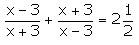17.

Solution: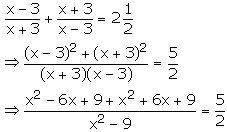2(2x2 + 18) = 5(x2 – 9)

4x2 + 36 = 5x2 – 45

x2 – 81 = 0

(x – 9)(x + 9) = 0

So, x – 9 = 0 or x + 9 = 0

Hence,

x = 9 or x = -9

Exercise 5(D) Page No: 59

1. Solve, each of the following equations, using the formula:

(i) x2 – 6x = 27

Solution:

Given equation, x2 – 6x = 27

x2 – 6x – 27 = 0

Here, a = 1 , b = -6 and c = -27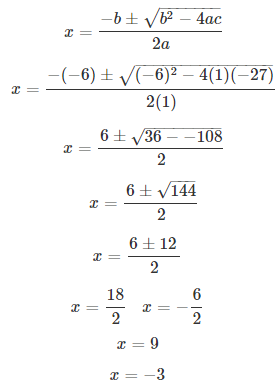Therefore, x = 9 or -3

(ii) x2 – 10x + 21 = 0

Solution:

Given equation, x2 – 10x + 21 = 0

Here, a = 1, b = -10 and c = 21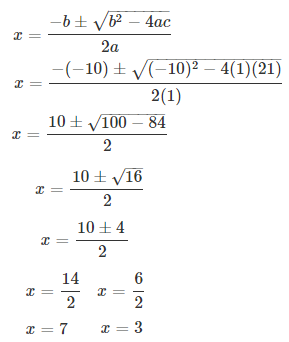Therefore, x = 7 or x = 3

(iii) x2 + 6x – 10 = 0

Solution:

Given equation, x2 + 6x – 10 = 0

Here, a = 1, b = 6 and c = -10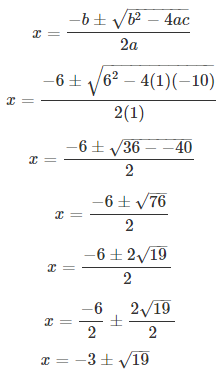Therefore, x = -3 + √19 or x = -3 – √19

(iv) x2 + 2x – 6 = 0

Solution:

Given equation, x2 + 2x – 6 = 0

Here, a = 1, b = 2 and c = -6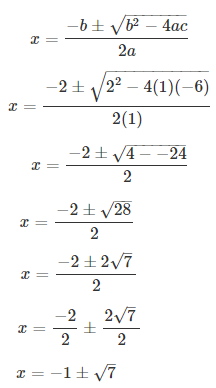Therefore, x = -1 + √7 or x = -1 – √7

(v) 3x2 + 2x – 1 = 0

Solution:

Given equation, 3x2 + 2x – 1 = 0

Here, a = 3, b = 2 and c = -1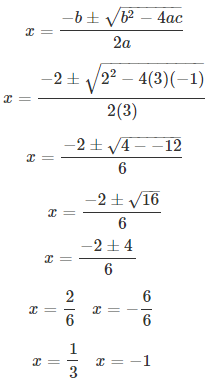Therefore, x = 1/3 or x = -1

(vi) 2x2 + 7x + 5 = 0

Solution:

Given equation, 2x2 + 7x + 5 = 0

Here, a = 2, b = 7 and c = 5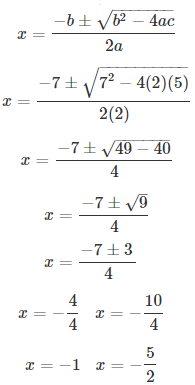Therefore, x = -1 or x = -5/2

(vii) 2/3 x = -1/6 x2 – 1/3

Solution:

Given equation, 2/3 x = -1/6 x2 – 1/3

1/6 x2 + 2/3 x + 1/3 = 0

Multiplying by 6 on both sides

x2 + 4x + 2 = 0

Here, a = 1, b = 4 and c = 2Therefore, x = -2 + √2 or x = -2 – √2

(viii) 1/15 x2 + 5/3 = 2/3 x

Solution:

Given equation, 1/15 x2 + 5/3 = 2/3 x

1/15 x2 – 2/3 x + 5/3 = 0

Multiplying by 15 on both sides

x2 – 10x + 25 = 0

Here, a = 1, b = -10 and c = 25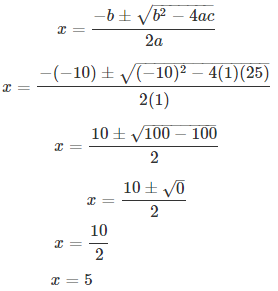Therefore, x = 5 (equal roots)

(ix) x2 – 6 = 2 √2 x

Solution:

Given equation, x2 – 6 = 2 √2 x

x2 – 2√2 x – 6 = 0

Here, a = 1, b = -2√2 and c = -6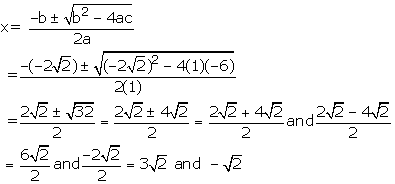Therefore, x = 3√2 or x = -√2

(x) 4/x – 3 = 5/ (2x + 3)

Solution:

Given equation, 4/x – 3 = 5/ (2x + 3)

(4 – 3x)/ x = 5/ (2x + 3)

On cross multiplying, we have

(4 – 3x)(2x + 3) = 5x

8x – 6x2 + 12 – 9x = 5x

6x2 + 6x – 12 = 0

Dividing by 6, we get

x2 + x – 2 = 0

Here, a = 1, b = 1 and c = -2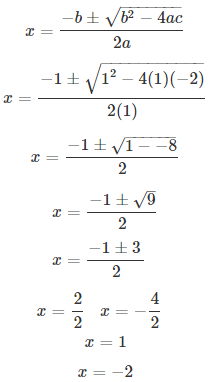Therefore, x = 1 or x = -2

(xi) 2x + 3/ x + 3 = x + 4/ x + 2

Solution:

Given equation, 2x + 3/ x + 3 = x + 4/ x + 2

On cross-multiplying, we have

(2x + 3) (x + 2) = (x + 4) (x + 3)

2x2 + 4x + 3x + 6 = x2 + 3x + 4x + 12

2x2 + 7x + 6 = x2 + 7x + 12

x2 + 0x – 6 = 0

Here, a = 1, b = 0 and c = -6

Therefore, x = √6 or x = -√6

(xii) √6x2 – 4x – 2√6 = 0

Solution:

Given equation, √6x2 – 4x – 2√6 = 0

Here, a = √6, b = -4 and c = -2√6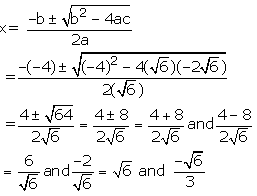Therefore, x = √6 or -√6/3

(xiii) 2x/ x – 4 + (2x – 5)/(x – 3) =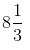Solution:

Given equation, 2x/ x – 4 + (2x – 5)/(x – 3) =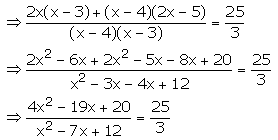25x2 – 175x + 300 = 12x2 – 57x + 60

13x2 – 118x + 240 = 0

Here, a = 13, b = -118 and c = 240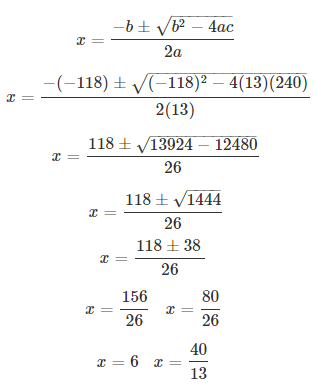Therefore, x = 6 or x = 40/13

(xiv)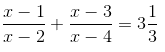Solution:

From the given equation,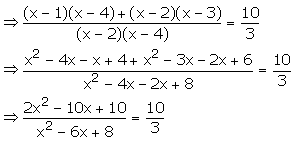10x2 – 60x + 80 = 6x2 – 30x + 30

4x2 – 30x + 50 = 0

2x2 – 15x + 25 = 0

Here, a = 2, b = -15 and c = 25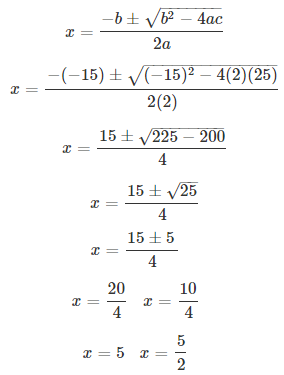Therefore, x = 5 or x = 5/2

2. Solve each of the following equations for x and give, in each case, your answer correct to one decimal place:

(i) x2 – 8x +5 = 0

(ii) 5x2 + 10x – 3 = 0

Solution:

(i) x2 – 8x + 5 = 0

Here, a = 1, b = -8 and c = 5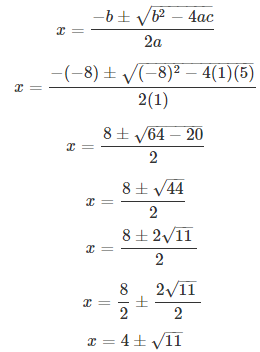x = 4 ± 3.3

Thus, x = 7.7 or x = 0.7

(ii) 5x2 + 10x – 3 = 0

Here, a = 5, b = 10 and c = -3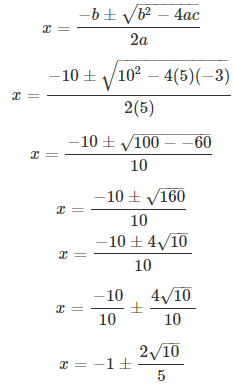Thus, x = 0.3 or x = -2.3

3. Solve each of the following equations for x and give, in each case, your answer correct to 2 decimal places:

(i) 2x2 – 10x + 5 = 0

Solution:

Given equation, 2x2 – 10x + 5 = 0

Here, a = 2, b = -10 and c = 5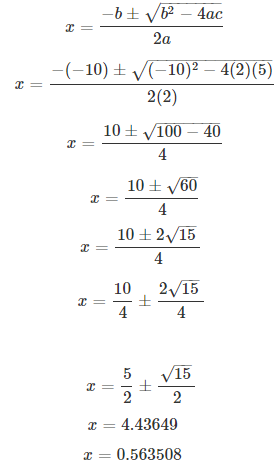Therefore, x = 4.44 or x = 0.56

(ii) 4x + 6/x + 13 = 0

Solution:

Given equation, 4x + 6/x + 13 = 0

Multiplying by x both sides, we get

4x2 + 13x + 6 = 0

Here, a = 4, b = 13 and c = 6Therefore, x = -0.56 or x = -2.70

(iii) 4x2 – 5x – 3 = 0

Solution:

Given equation, 4x2 – 5x – 3 = 0

Here, a = 4, b = -5 and c = -3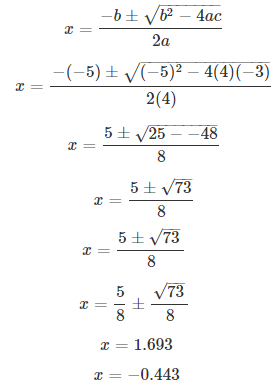Therefore, x = 1.70 or x = -0.44

(iv) x2 – 3x – 9 = 0

Solution:

Given equation, x2 – 3x – 9 = 0

Here, a = 1, b = -3 and c = -9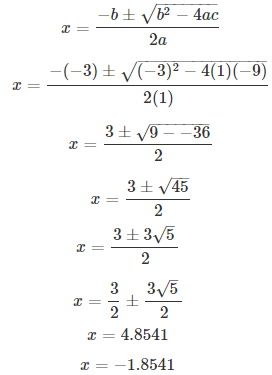Therefore, x = 4.85 or x = -1.85

(v) x2 – 5x – 10 = 0

Solution:

Given equation, x2 – 5x – 10 = 0

Here, a = 1, b = -5 and c = -10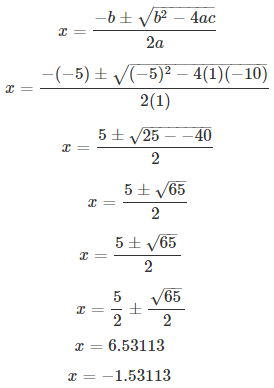Therefore, x = 6.53 or x = -1.53

4. Solve each of the following equations for x and give, in each case, your answer correct to 3 decimal places:

(i) 3x2 – 12x – 1 = 0

(ii) x2 – 16 x +6 = 0

(iii) 2x2 + 11x + 4 = 0

Solution:

(i) Given equation, 3x2 – 12x – 1 = 0

Here, a = 3, b = -12 and c = -1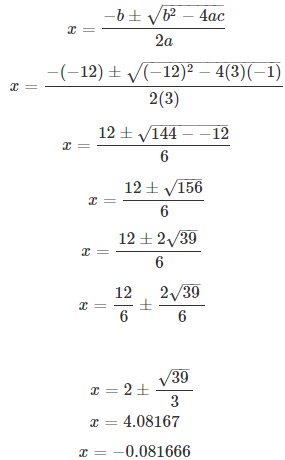Therefore, x = 4.082 or x = -0.082

(ii) Given equation, x2 – 16 x + 6 = 0

Here, a = 1, b = -16 and c = 6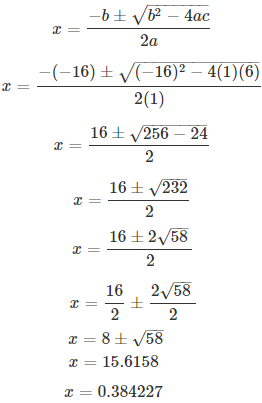Therefore, x = 15.616 or x = 0.384

(iii) Given equation, 2x2 + 11x + 4 = 0

Here, a = 2, b = 11 and c = 4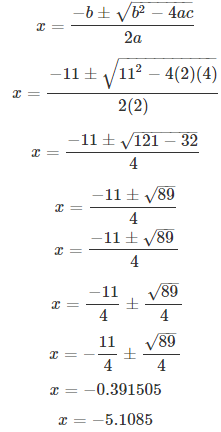Therefore, x = -0.392 or x = -5.110

5. Solve:

(i) x4 – 2x2 – 3 = 0

Solution:

Given equation, x4 – 2x2 – 3 = 0

x4 – 3x2 + x2 – 3 = 0

x2(x2 – 3) + 1(x2 – 3) = 0

(x2 + 1) (x2 – 3) = 0

So, x2 + 1 = 0 (which is not possible) or x2 – 3 = 0

Hence,

x2 – 3 = 0

x = ± √3

(ii) x4 – 10x2 + 9 = 0

Solution:

Given equation, x4 – 10x2 + 9 = 0

x4 – x2 – 9x2+ 9 = 0

x2(x2 – 1) – 9(x2 – 1) = 0

(x2 – 9)(x2 – 1) = 0

So, we have

x2 – 9 = 0 or x2 – 1 = 0

Hence,

x = ± 3 or x = ± 1

Exercise 5(E) Page No: 66

1. Solve each of the following equations: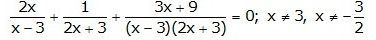Solution:

Given equation,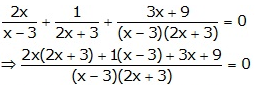4x2 + 6x + x – 3 + 3x + 9 = 0

4x2 + 10x + 6 = 0

4x2 + 4x + 6x + 6 = 0

4x(x + 1) + 6(x + 1) = 0

(4x + 6) (x + 1) = 0

So, 4x + 6 = 0 or x + 1 = 0

x = -1 or x = -6/4 = -3/2 (rejected as this value is excluded in the domain)

Therefore,

x = -1 is the only solution

2. (2x + 3)2 = 81

Solution:

Given, (2x + 3)2 = 81

Taking square root on both sides we have,

2x + 3 = ± 9

2x = ± 9 – 3

x = (± 9 – 3)/ 2

So,

x = (9 – 3)/ 2 or (-9 – 3)/ 2

Therefore,

x = 3 or x = -6

3. a2x2 – b2 = 0

Solution:

Given equation, a2x2 – b2 = 0

(ax)2 – b2 = 0

(ax + b)(ax – b) = 0

So,

ax + b = 0 or ax – b = 0

Therefore,

x = -b/a or b/a

4. x2 – 11/4 x + 15/8 = 0

Solution:

Given equation, x2 – 11/4 x + 15/8 = 0

Taking L.C.M we have,

(8x2 – 22x + 15)/ 8 = 0

8x2 – 22x + 15 = 0

8x2 – 12x – 10x + 15 = 0

4x(2x – 3) – 5(2x – 3) = 0

(4x – 5)(2x – 3) = 0

So, 4x – 5 = 0 or 2x – 3 = 0

Therefore,

x = 5/4 or x = 3/2

5. x + 4/x = -4; x ≠ 0

Solution:

Given equation, x + 4/x = -4

(x2 + 4)/ x = -4

x2 + 4 = -4x

x2 + 4x + 4 = 0

x2 + 2x + 2x + 4 = 0

x(x + 2) + 2(x + 2) = 0

(x + 2)(x + 2) = 0

(x + 2)2 = 0

Taking square – root we have,

x + 2 = 0

Therefore, x = -2

6. 2x4 – 5x2 + 3 = 0

Solution:

Given equation, 2x4 – 5x2 + 3 = 0

Let’s take x2 = y

Then, the equation becomes

2y2 – 5y + 3 = 0

2y2 – 2y – 3y + 3 = 0

2y(y – 1) – 3(y – 1) = 0

(2y – 3) (y – 1) = 0

So, 2y – 3 = 0 or y – 1 = 0

y = 3/2 or y = 1

And, we have taken y = x2

Thus,

x2 = 3/2 or x2 = 1

x = ± √(3/2) or x = ±1

7. x4 – 2x2 – 3 = 0

Solution:

Given equation, x4 – 2x2 – 3 = 0

Let’s take x2 = y

Then, the equation becomes

y2 – 2y – 3 = 0

y2 – 3y + y – 3 = 0

y(y – 3) + 1(y – 3) = 0

(y + 1)(y – 3) = 0

So, y + 1 = 0 or y – 3 = 0

y = -1 or y = 3

And, we have taken y = x2

Thus,

x2 = – 1(impossible, no real solution)

x2 = 3

x = ± √3

8.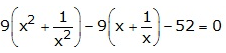Solution:

Let us take (x + 1/x) = y …. (1)

Now, squaring it on both sides

(x + 1/x)2 = y2

x2 + 1/x2 + 2 = y2

So,

x2 + 1/x2 = y2 – 2 ….. (2)

Using (1) and (2) in the given equation, we have

9(y2 – 2) – 9(y) – 52 = 0

9y2 – 18 – 9y – 52 = 0

9y2 – 9y – 70 = 0

9y2 – 30y + 21y – 70 = 0

3y(3y – 10) + 7(3y – 10) = 0

(3y + 7)(3y – 10) = 0

So, 3y + 7 = 0 or 3y – 10 = 0

y = -7/3 or y = 10/3

Now,

x + 1/x = -7/3 or x + 1/x = 10/3

(x2 + 1)/x = -7/3 or (x2 + 1)/x = 10/3

3x2 – 10x + 3 = 0 or 3x2 + 7x + 3 = 0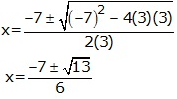3x2 – 9x – x + 3 = 0 or

3x(x – 3) – 1(x – 3) = 0

(3x – 1)(x – 3) = 0

So, x = 1/3 or 3

9.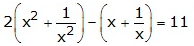Solution:

Let us take (x + 1/x) = y …. (1)

Now, squaring it on both sides

(x + 1/x)2 = y2

x2 + 1/x2 + 2 = y2

So,

x2 + 1/x2 = y2 – 2 ….. (2)

Using (1) and (2) in the given equation, we have

2(y2 – 2) – (y) = 11

2y2 – 4 – y = 11

2y2 – y – 15 = 0

2y2 – 6y + 5y – 15 = 0

2y(y – 3) + 5(y – 3) = 0

(2y + 5) (y – 3) = 0

So,

2y + 5 = 0 or y – 3 = 0

y = -5/2 or y = 3

Now,

x + 1/x = -5/2 or x + 1/x = 3

(x2 + 1)/x = -5/2 or (x2 + 1)/x = 3

2(x2 + 1) = -5x or x2 + 1 = 3x

2x2 + 5x + 2 = 0 or x2 – 3x + 1 = 0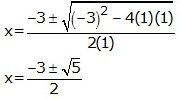2x2 + 4x + x + 2 = 0 or

2x(x + 2) + 1(x + 2) = 0

(2x + 1)(x + 2) = 0

Hence, x = -1/2 or -2

10.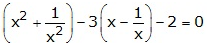Solution:

Let us take (x – 1/x) = y …. (1)

Now, squaring it on both sides

(x – 1/x)2 = y2

x2 + 1/x2 – 2 = y2

So,

x2 + 1/x2 = y2 + 2 ….. (2)

Using (1) and (2) in the given equation, we have

(y2 + 2) – 3(y) – 2 = 0

y2 -3y = 0

y(y – 3) = 0

So, y = 0 or y – 3 = 0

Now,

(x – 1/x) = 0 or (x – 1/x) = 3

x2 – 1 = 0 or x2 – 1 = 3x

x2 = 1 or x2 – 3x – 1 = 0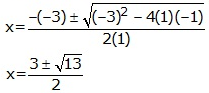Therefore,

x = ± 1 or

Exercise 5(F) Page No: 67

1. Solve:

(i) (x + 5) (x – 5) = 24

Solution:

Given equation, (x + 5) (x – 5) = 24

x2 – 25 = 24

x2 = 49

Thus,

x = ± 7

(ii) 3x2 – 2√6x + 2 = 0

Solution:

Given equation, 3x2 – 2√6x + 2 = 0

3x2 – √6x – √6x + 2 = 0

√3x(√3x – √2) – √2(√3x – √2) = 0

(√3x – √2) (√3x – √2) = 0

So, √3x – √2 = 0 or √3x – √2 = 0

Therefore,

x = √(2/3), √(2/3) (equal roots)

(iii) 3√2x2 – 5x – √2 = 0

Solution:

Given equation, 3√2x2 – 5x – √2 = 0

3√2x2 – 6x + x – √2 = 0

3√2x(x – √2) + 1(x – √2) = 0

(3√2x + 1) (x – √2) = 0

So, 3√2x + 1 = 0 or x – √2 = 0

Therefore,

x = -1/ 3√2 or x = √2

(iv) 2x – 3 = √(2x2 – 2x + 21)

Solution:

Given equation, 2x – 3 = √(2x2 – 2x + 21)

On squaring on both sides, we have

(2x – 3)2 = 2x2 – 2x + 21

4x2 + 9 – 12x = 2x2 – 2x + 21

2x2 – 10x – 12 = 0

Dividing by 2, we get

x2 – 5x – 6 = 0

x2 – 6x + x – 6 = 0

x(x – 6) + 1(x – 6) = 0

(x + 1) (x – 6) = 0

So, x + 1 = 0 or x – 6 = 0

Thus, we get

x = -1 or x = 6

But, putting x = -1 the L.H.S become negative. And we know that the square root function always gives a positive value.

Therefore,

x = 6 is the only solution.

2. One root of the quadratic equation 8x2 + mx + 15 = 0 is ¾. Find the value of m. Also, find the other root of the equation.

Solution:

Given equation, 8x2 + mx + 15 = 0

One of the roots is ¾, and hence it satisfies the given equation

So,

8(3/4)2 + m(3/4) + 15 = 0

8(9/16) + m(3/4) + 15 = 0

18/4 + 3m/4 + 15 = 0

Taking L.C.M, we have

(18 + 3m + 60)/4 = 0

18 + 3m + 60 = 0

3m = – 78

m = -26

Now, putting the value of m in the given equation, we get

8x2 + (-26)x + 15 = 0

8x2 – 26x + 15 = 0

8x2 – 20x – 6x + 15 = 0

4x(2x – 5) – 3(2x – 5) = 0

(4x – 3) (2x – 5) = 0

So, 4x – 3 = 0 or 2x – 5 = 0

Therefore.

x = ¾ or x = 5/2

3. Show that one root of the quadratic equation x2 + (3 – 2a)x – 6a = 0 is -3. Hence, find its other root.

Solution:

Given quadratic equation, x2 + (3 – 2a)x – 6a = 0

Now, putting x = -3 we have

(-3)2 + (3 – 2a)( -3) – 6a = 0

9 – 9 + 6a – 6a = 0

0 = 0

Since, x = -3 satisfies the given equation -3 is one of the root of the quadratic equation.

x2 + (3 – 2a)x – 6a = 0

x2 + 3x – 2ax – 6a = 0

x(x + 3) – 2a(x + 3) = 0

(x – 2a) (x + 3) = 0

So, x – 2a = 0 or x + 3 =0

x = 2a or x = -3

Hence, the other root is 2a.

4. If p – 15 = 0 and 2x2 + px + 25 = 0: find the values of x.

Solution:

Given equations, p – 15 = 0 and 2x2 + px + 25 = 0

Thus, p = 15

Now, using p in the quadratic equation, we get

2x2 + (15)x + 25 = 0

2x2 + 10x + 5x + 25 = 0

2x(x + 5) + 5(x + 5) = 0

(2x + 5) (x + 5) = 0

So, 2x + 5 = 0 or x + 5 = 0

Hence,

x = -5/2 or x = -5

5. Find the solution of the quadratic equation 2x2 – mx – 25n = 0; if m + 5 = 0 and n – 1 = 0.

Solution:

Given,

m + 5 = 0 and n – 1 = 0

so,

m = -5 and n = 1

Now, putting these values in the given quadratic equation 2x2 – mx – 25n = 0, we get

2x2 – (-5)x – 25(1) = 0

2x2 + 5x – 25 = 0

2x2 + 10x – 5x – 25 = 0

2x(x + 5) -5(x + 5) = 0

(2x – 5) (x + 5) = 0

So, 2x – 5 = 0 or x + 5 = 0

Hence,

x = 5/2 or x = -5

6. If m and n are roots of the equation: 1/x – 1/(x-2) = 3: where x ≠ 0 and x ≠ 2; find m x n.

Solution:

Given equation, 1/x – 1/(x-2) = 3

(x – 2 – x)/ (x(x – 2)) = 3

-2 = 3(x2 – 2x)

3x2 – 6x + 2 = 0

Solving by using quadratic formula, we get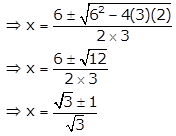And, since m and n are roots of the equation, we have

m = (√3 + 1)/ √3 n = (√3 – 1)/ √3

So,

m x n = (√3 + 1)/ √3 x (√3 – 1)/ √3 = [(√3)2 – 1]/ (√3)2

Thus,

m x n = 2/3

The given solutions are as per the 2019-20 Concise Selina textbook. The Selina Solutions for the academic year 2020-21 will be updated soon.